# Concise Selina Solutions for Class 9 Maths Chapter 17- Circles

Selina Solutions are useful for students as it helps them in scoring high marks in the examination. The Selina Solutions contain detailed step-by-step explanation of all the problems that come under Chapter 17, Circles, of the Class 9 Selina Textbook.

These solutions are prepared by subject matter experts at BYJU’S, describing the complete method of solving problems. By understanding the concepts used in Selina Solutions for Class 9 Maths, students will be able to clear all their doubts related to “Circles”.

## Download PDF of Selina Solutions for Class 9 Maths Chapter 17:-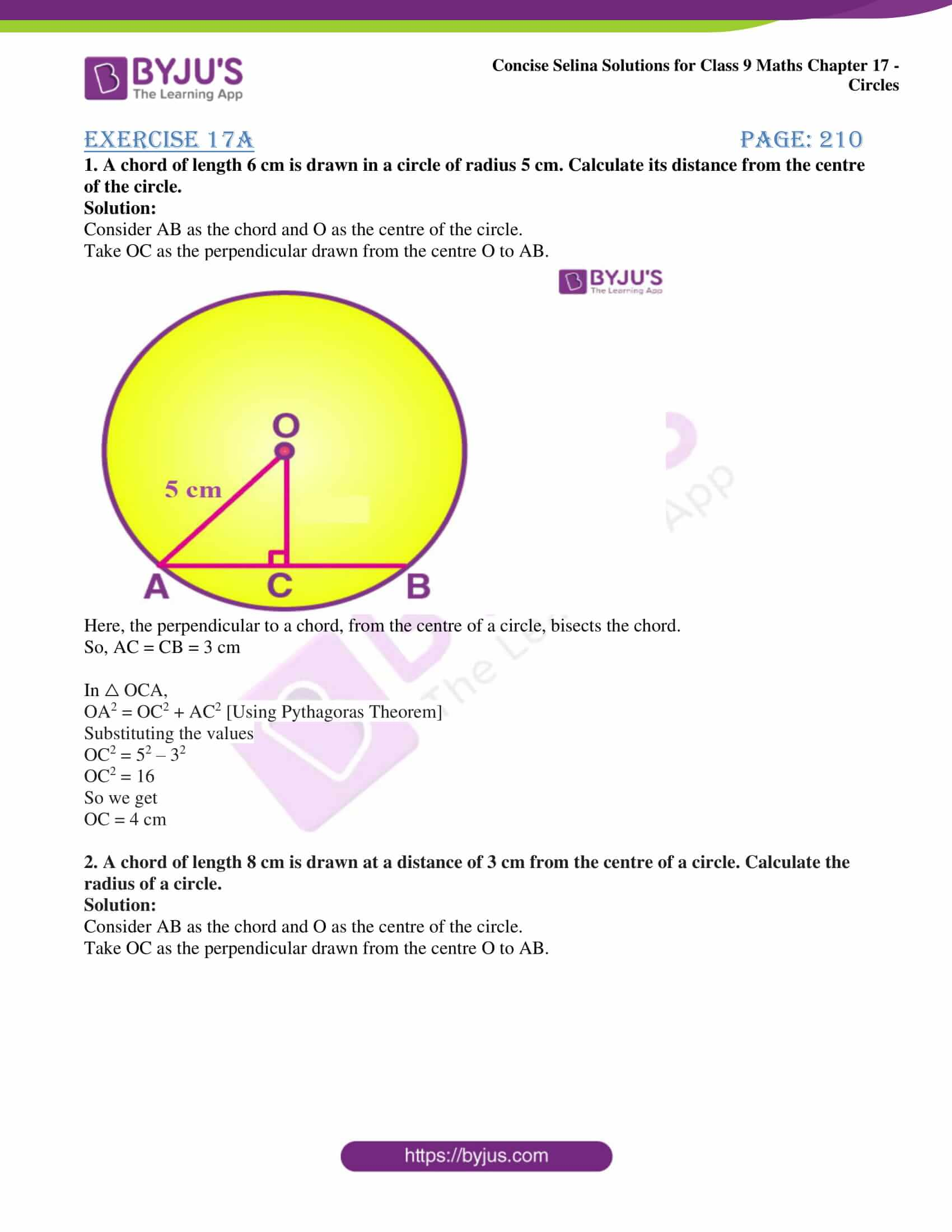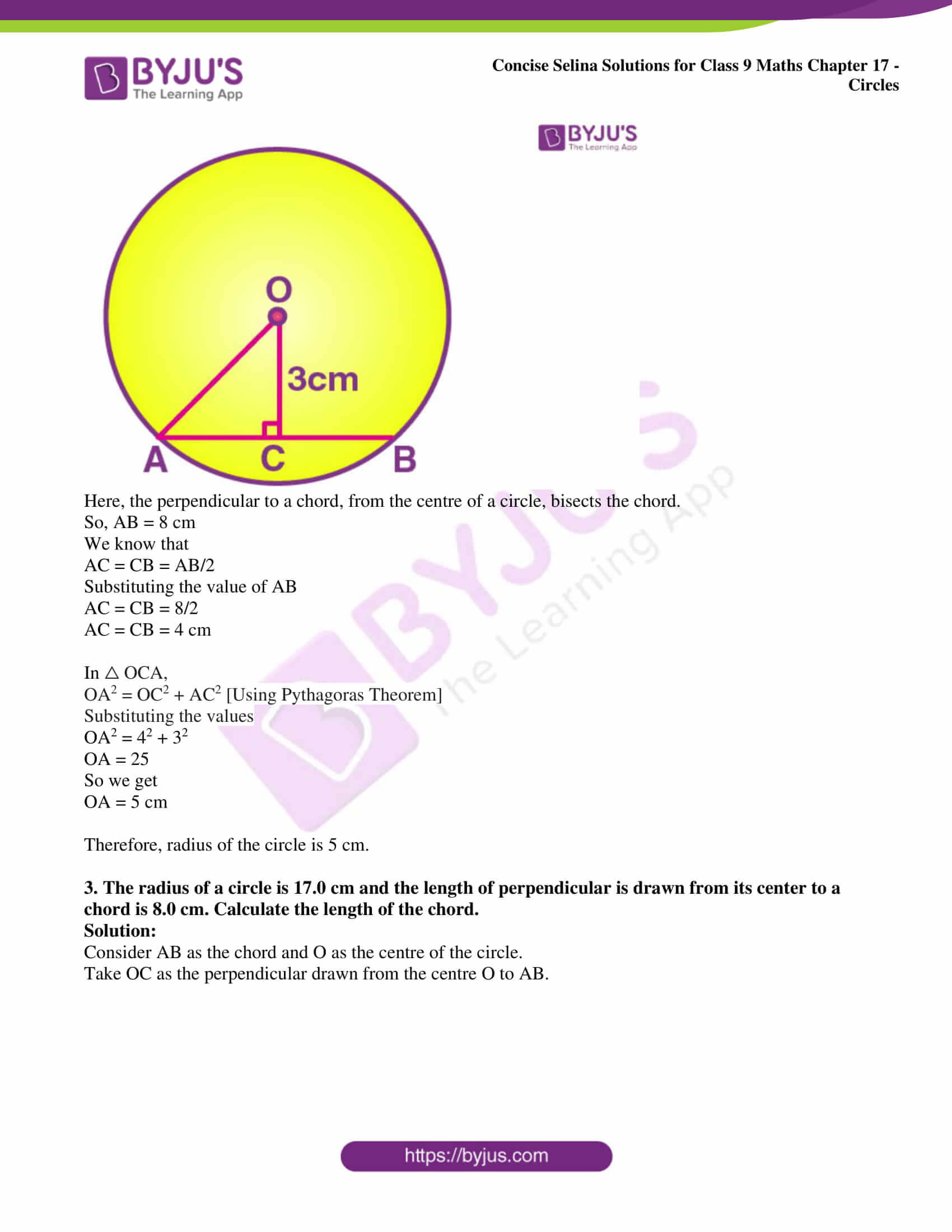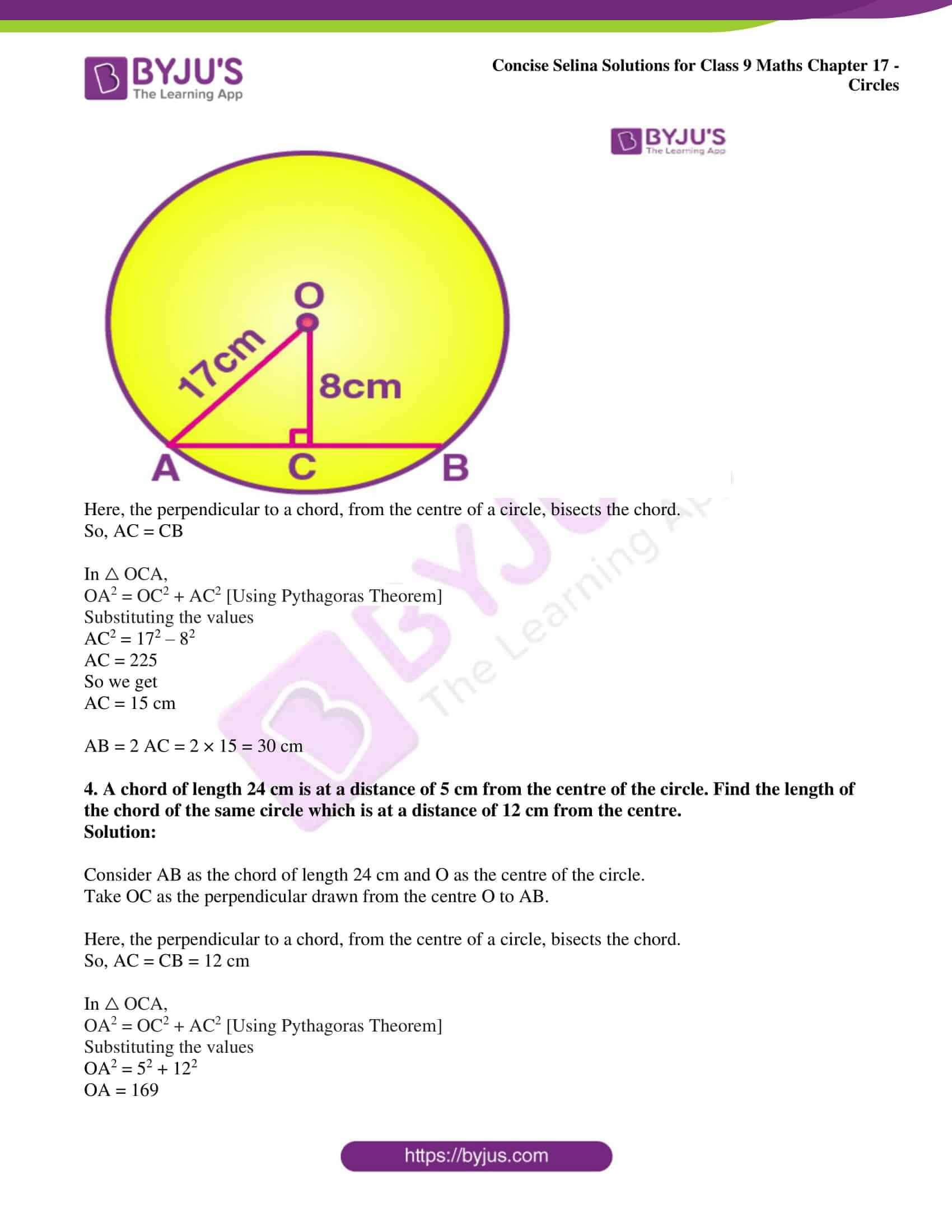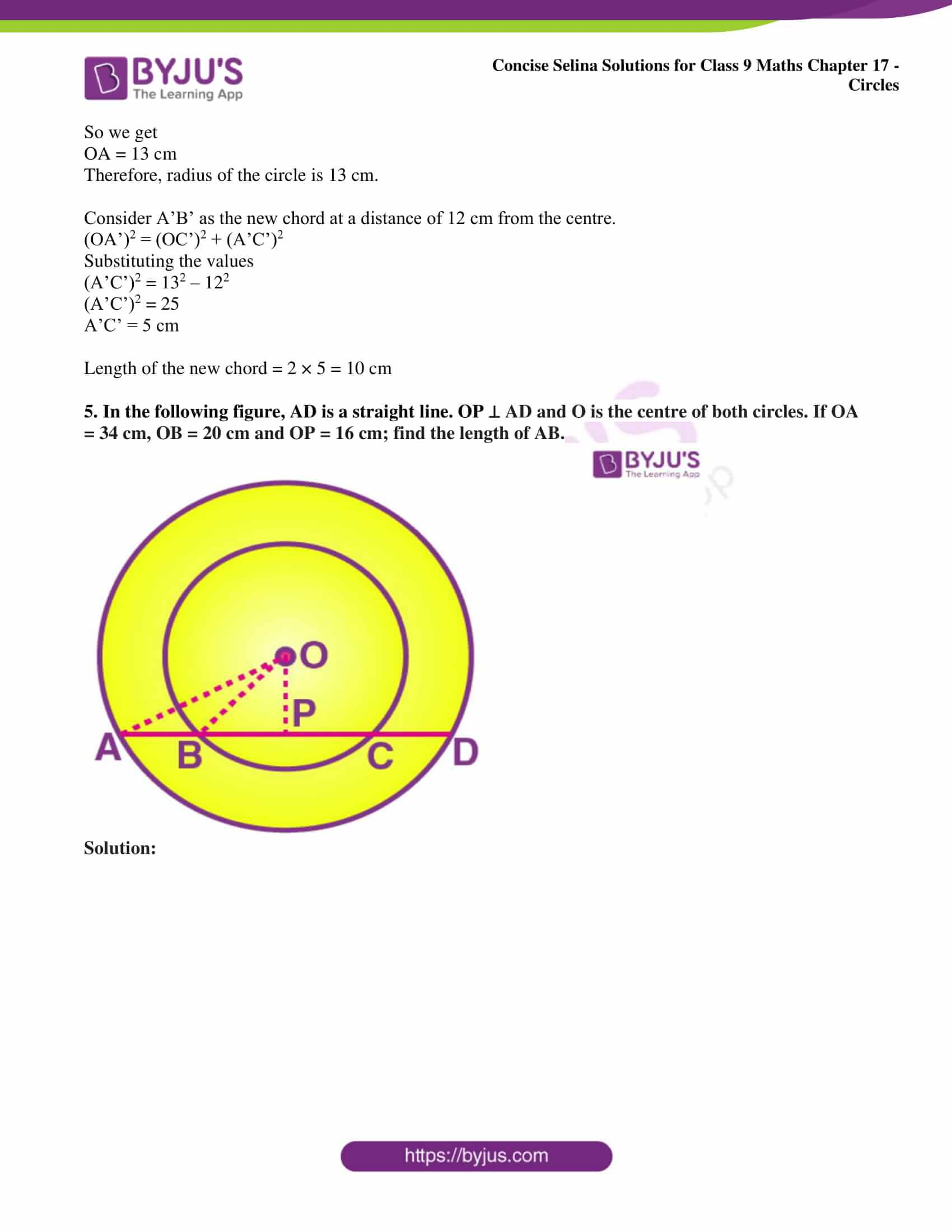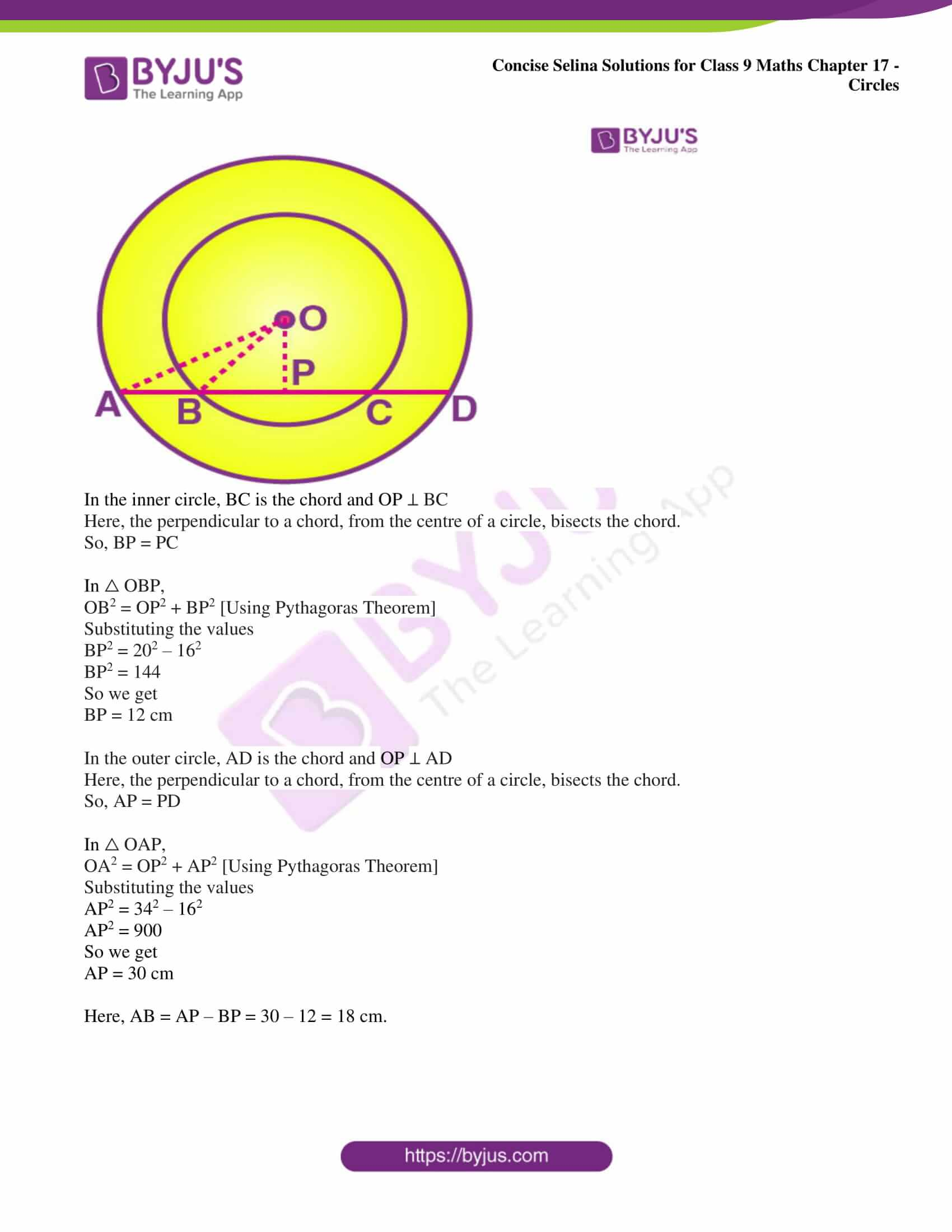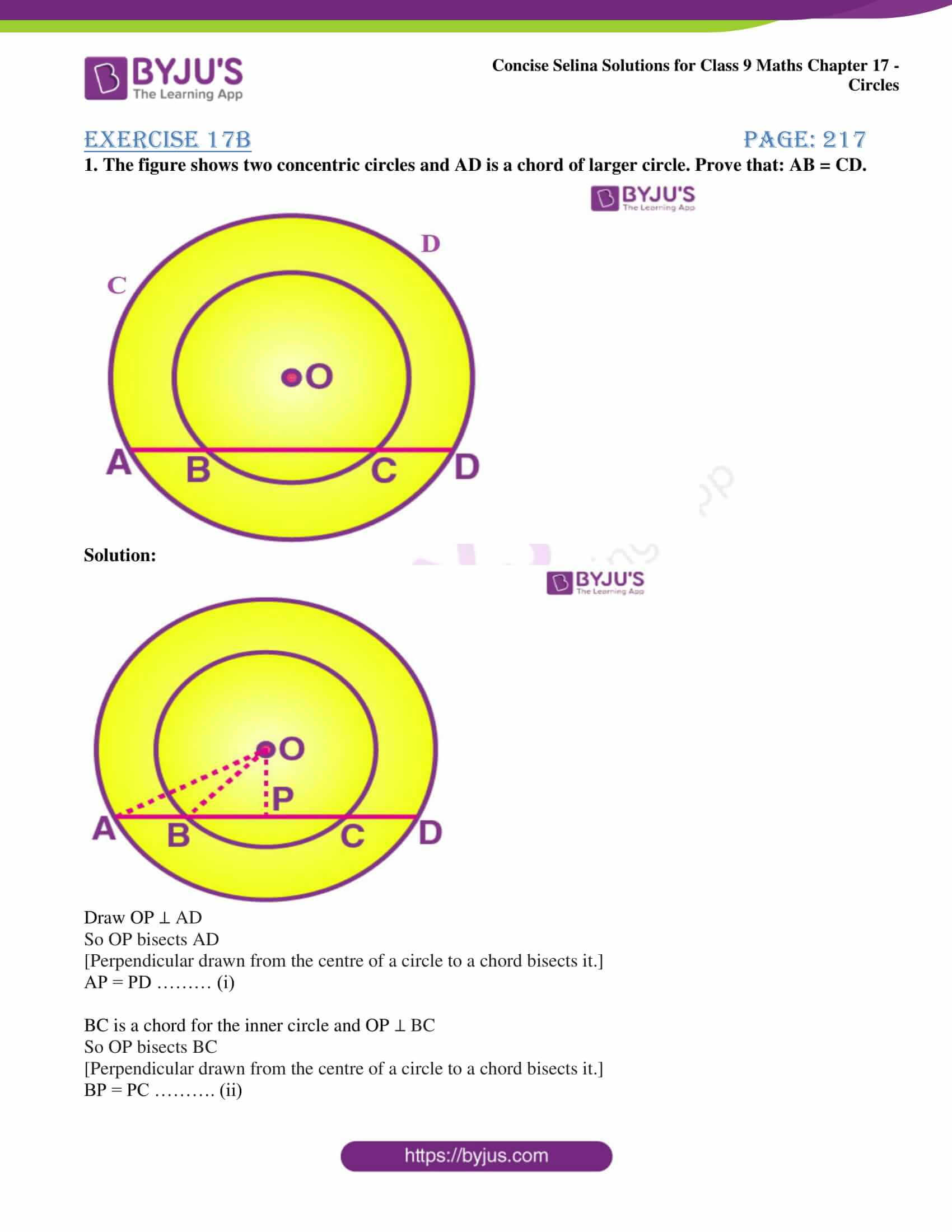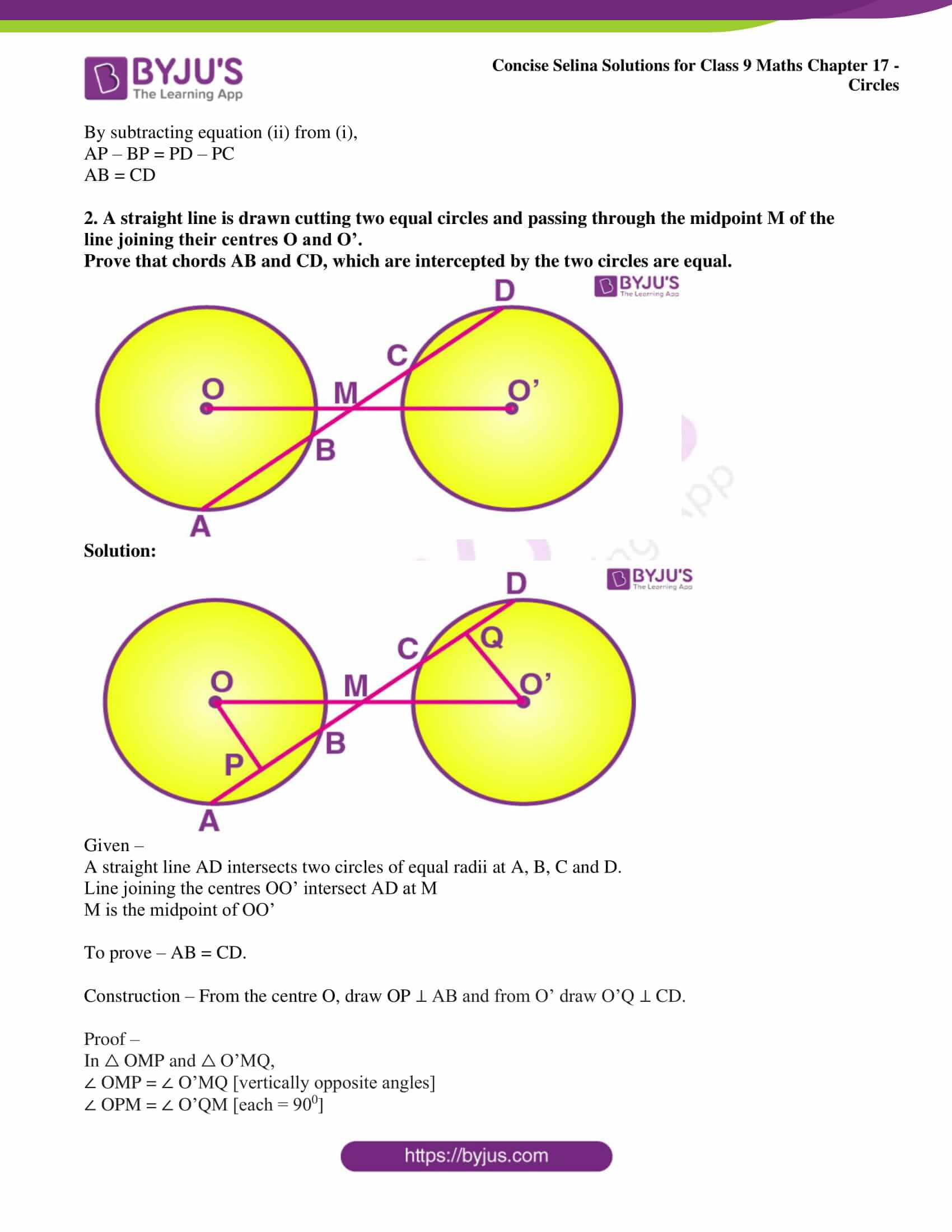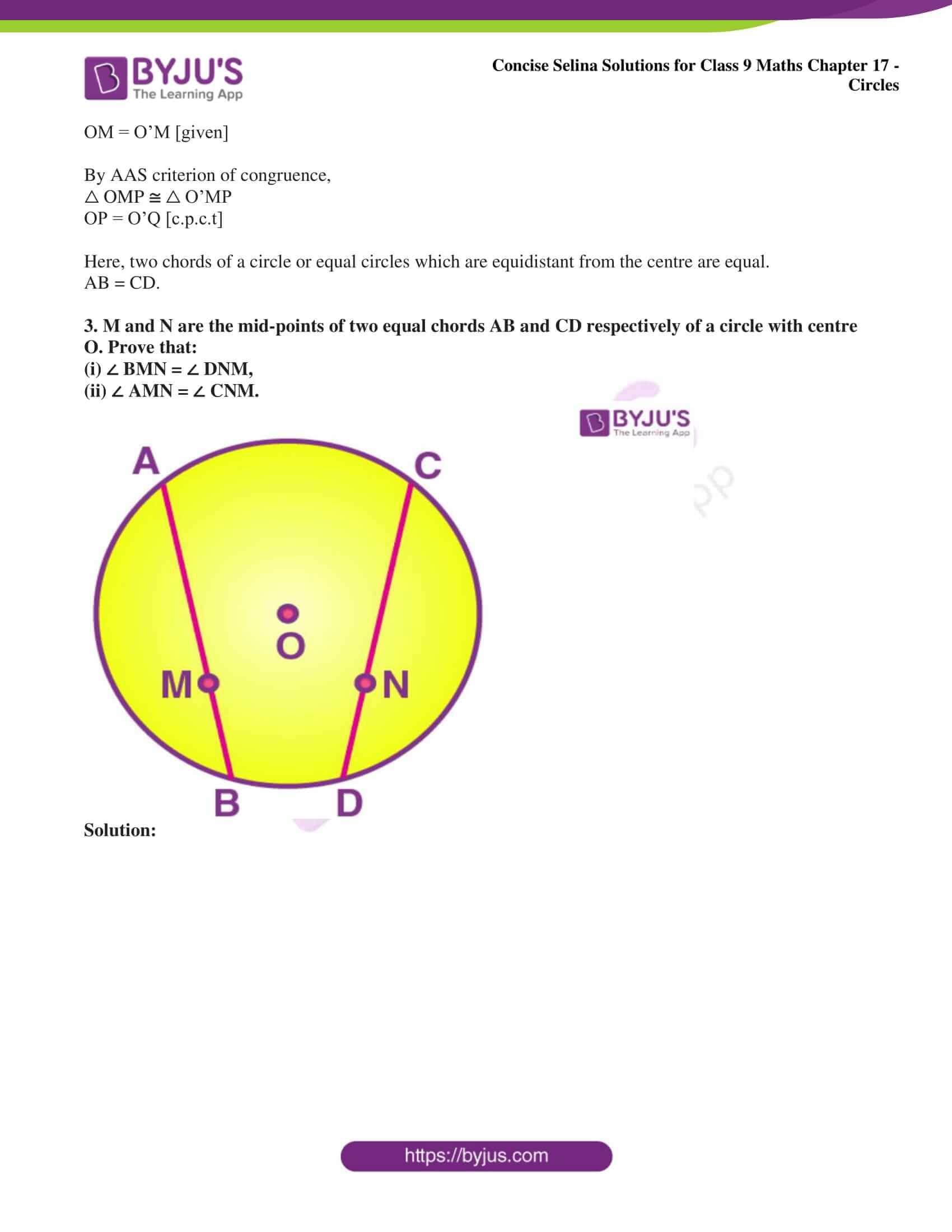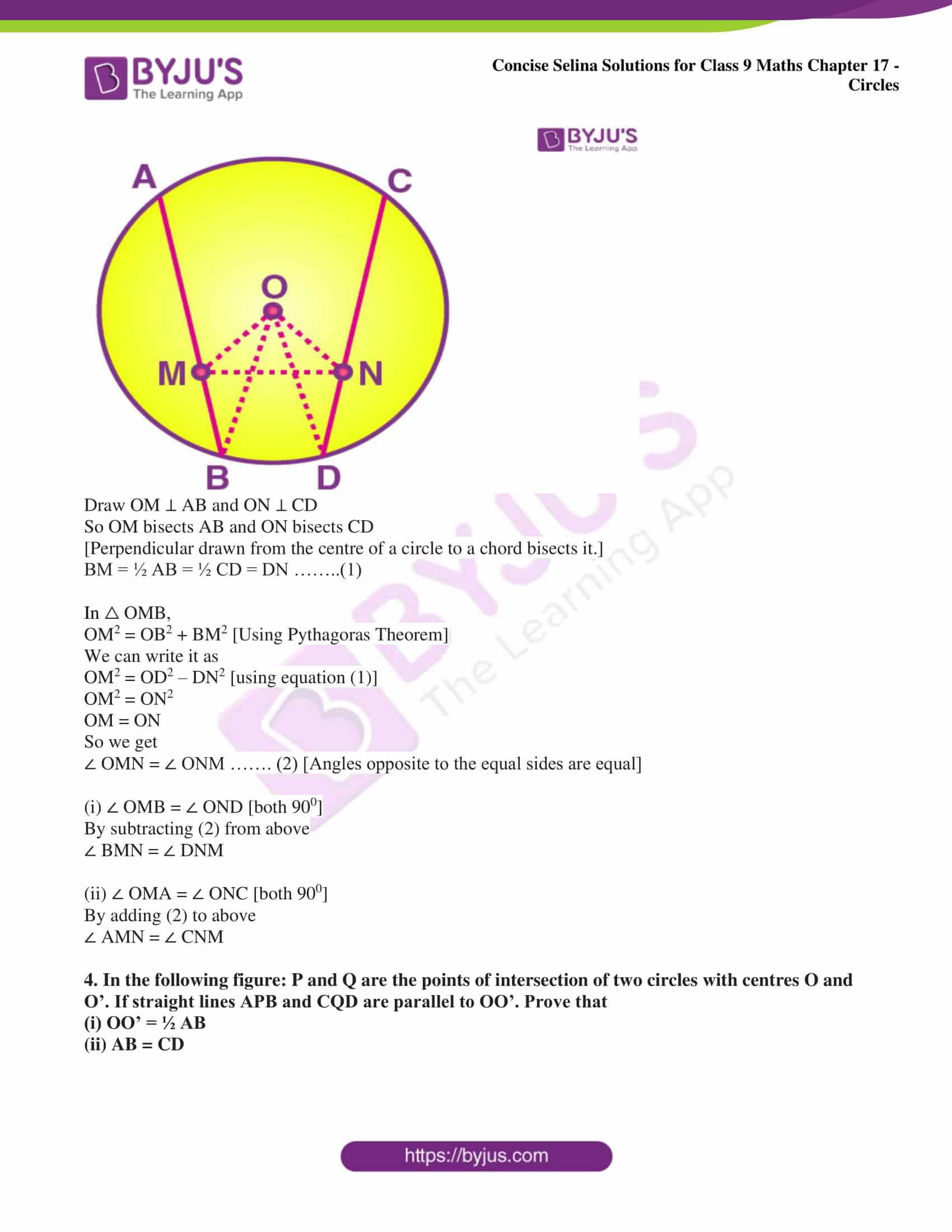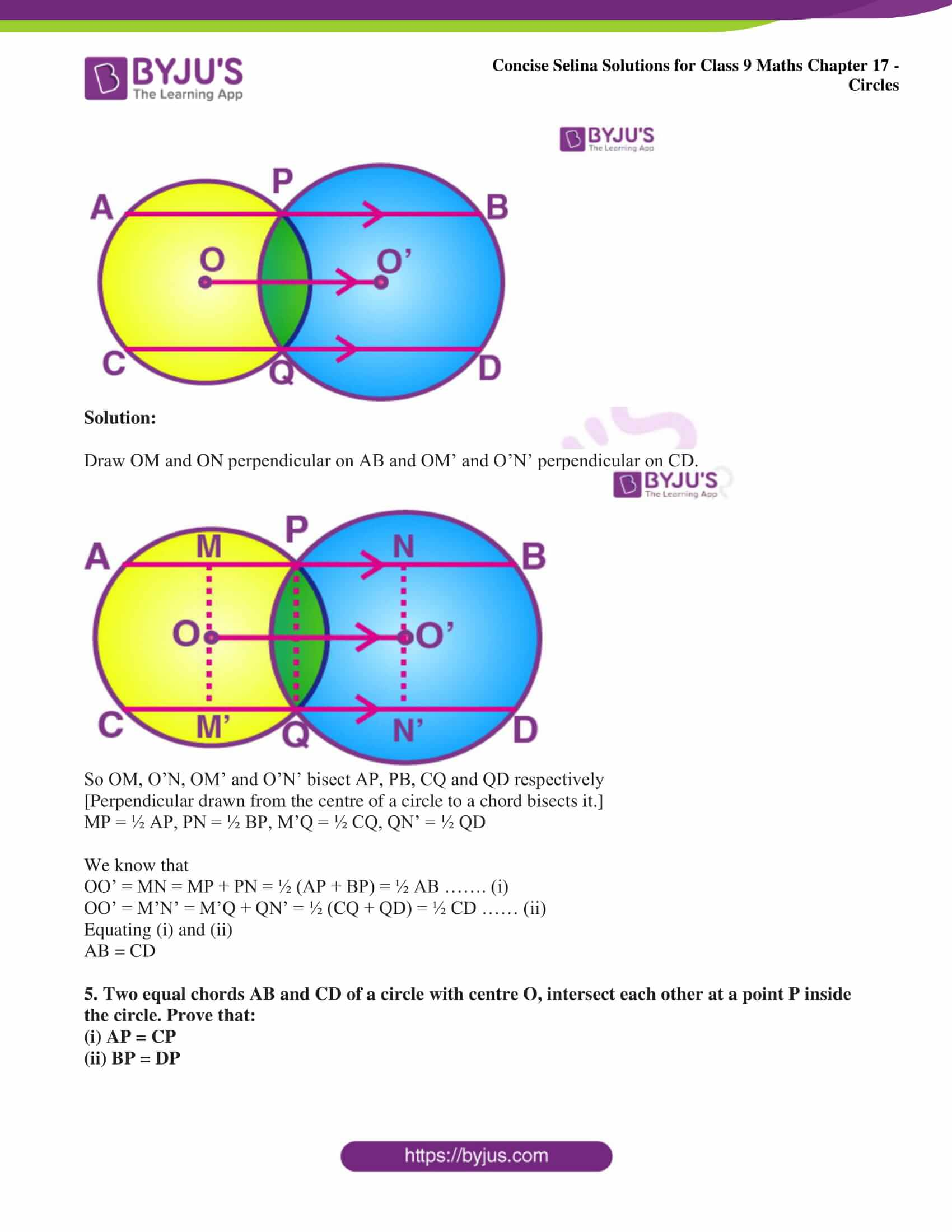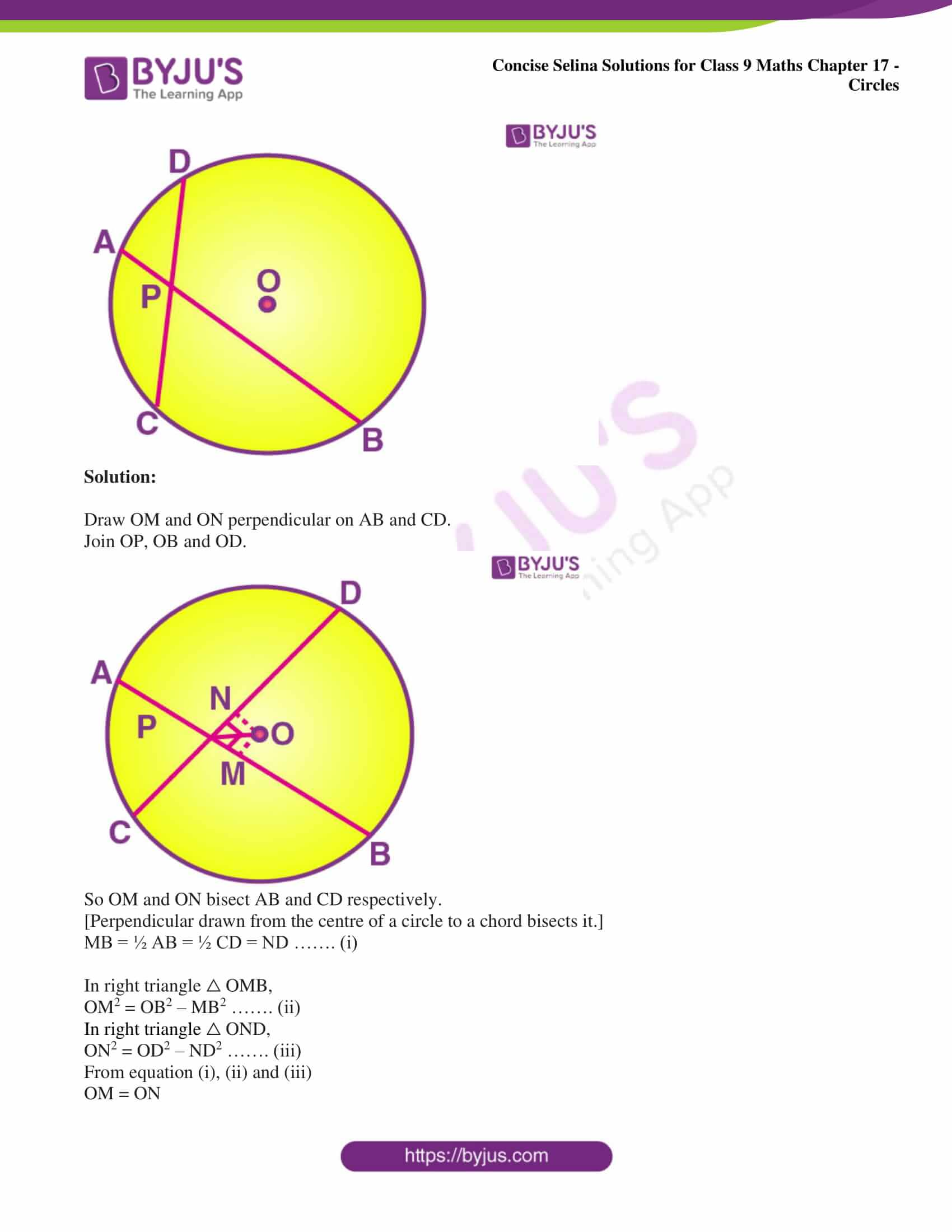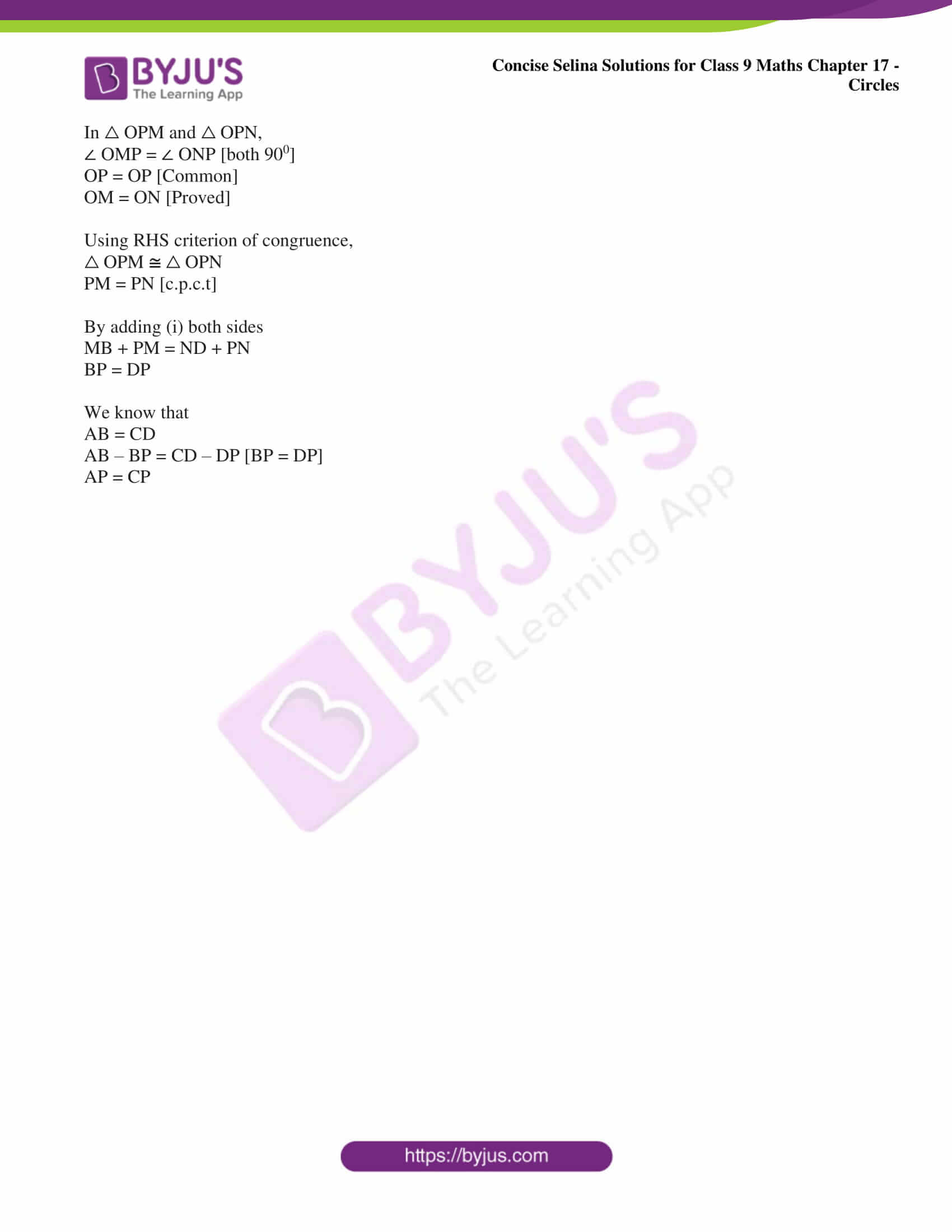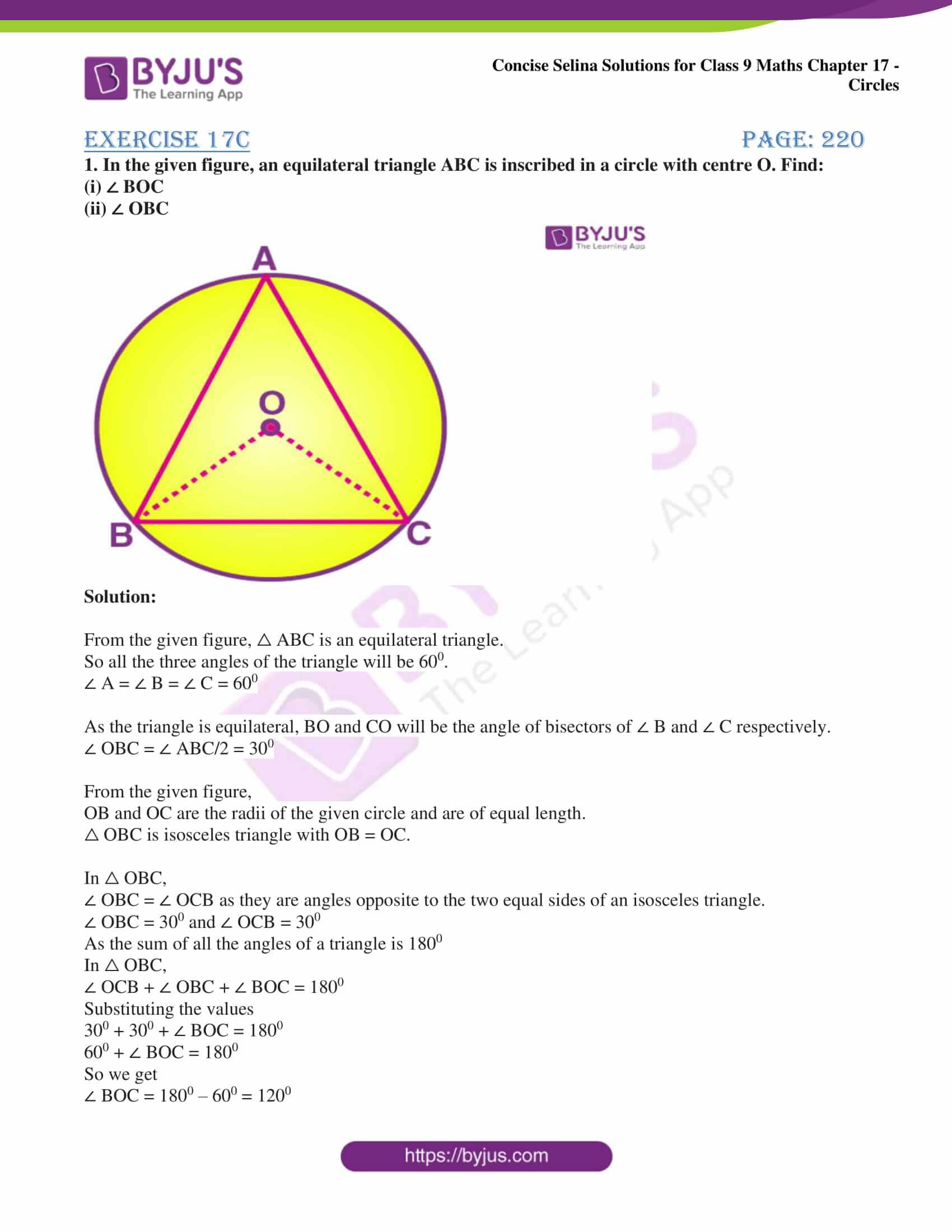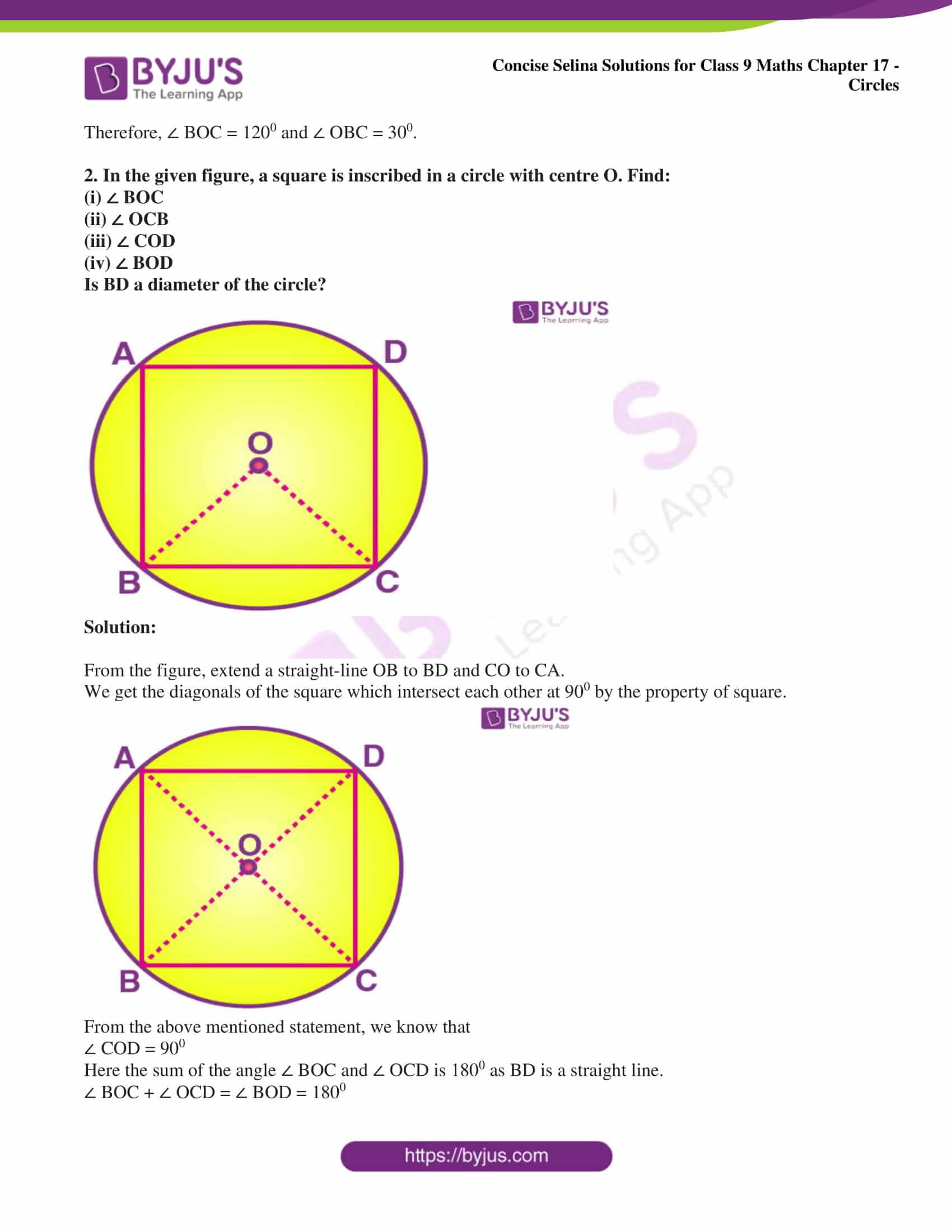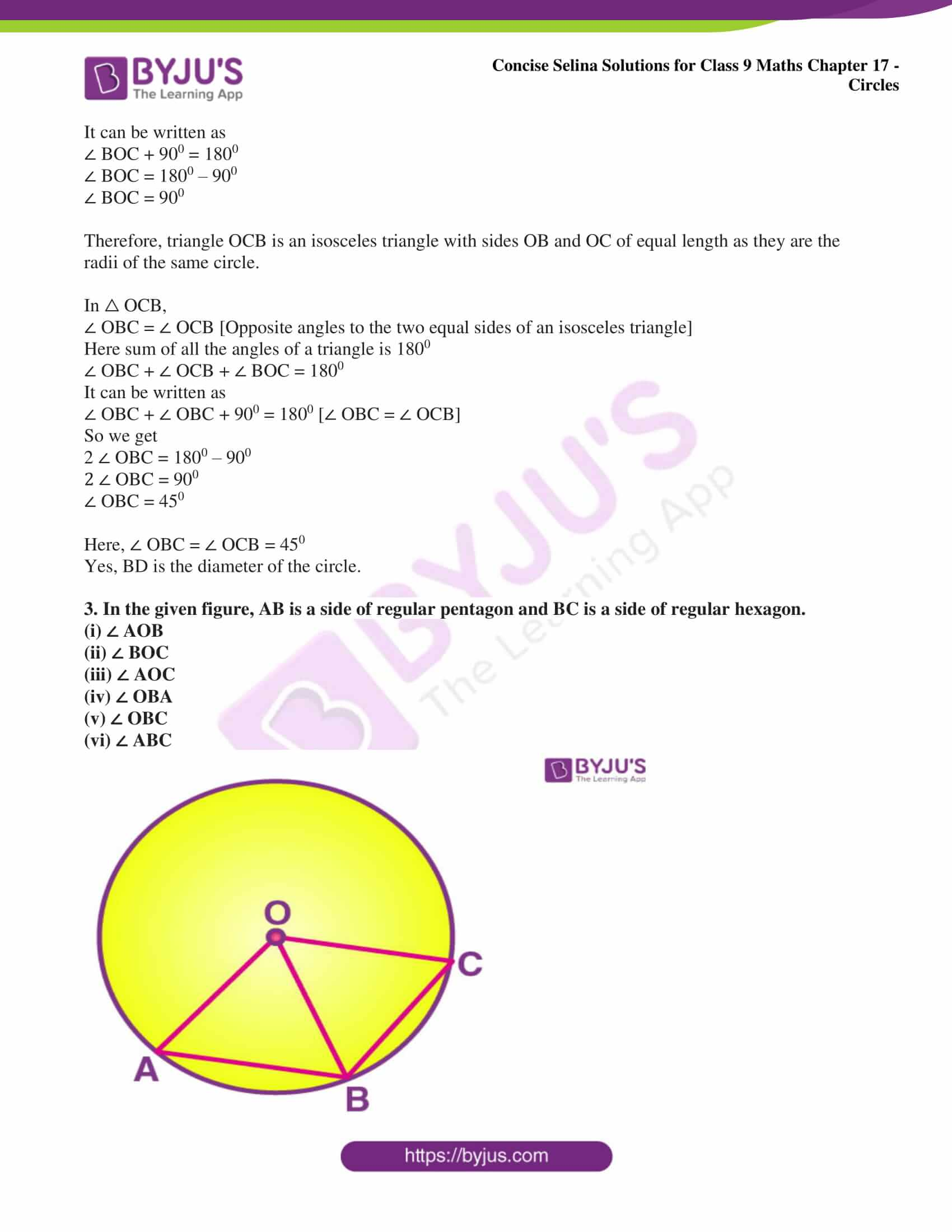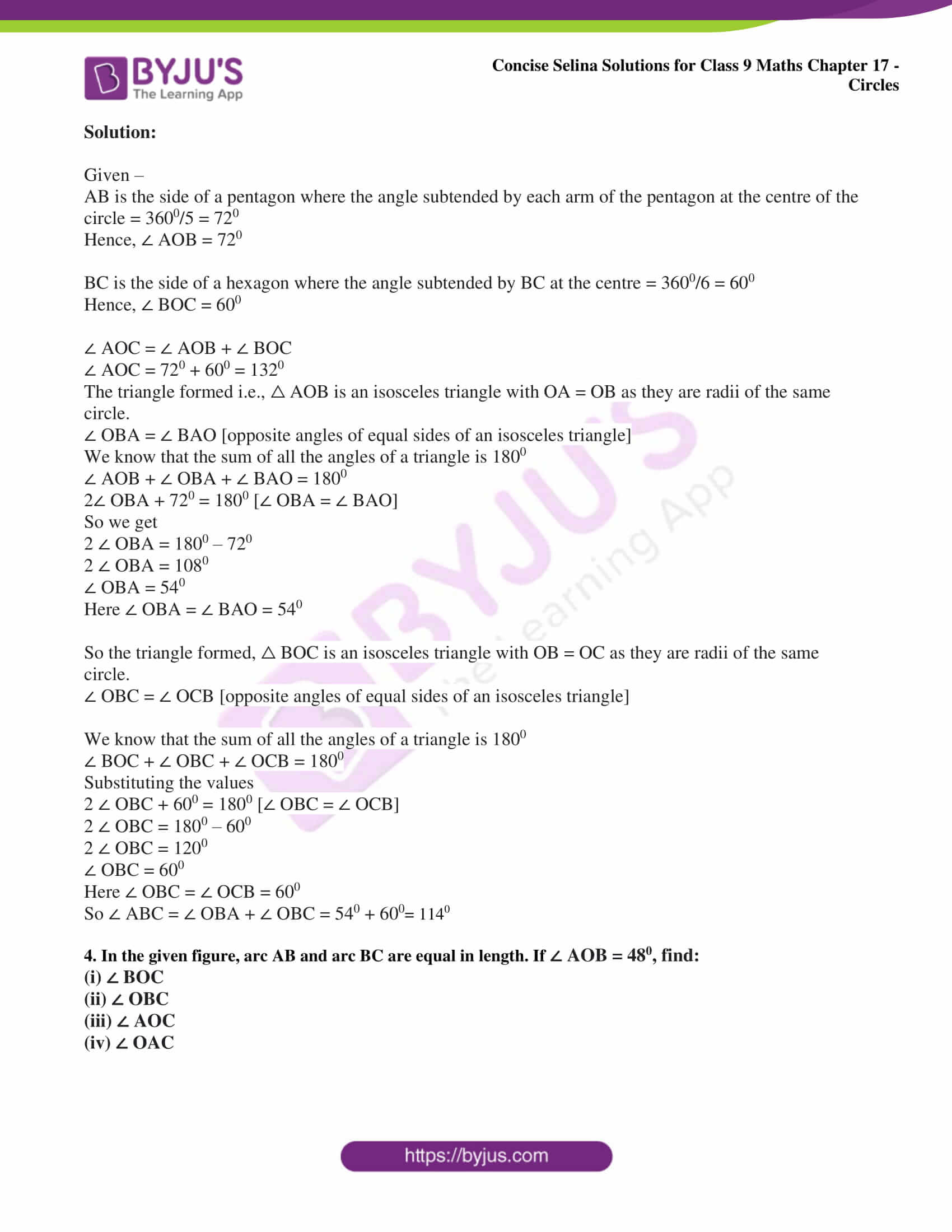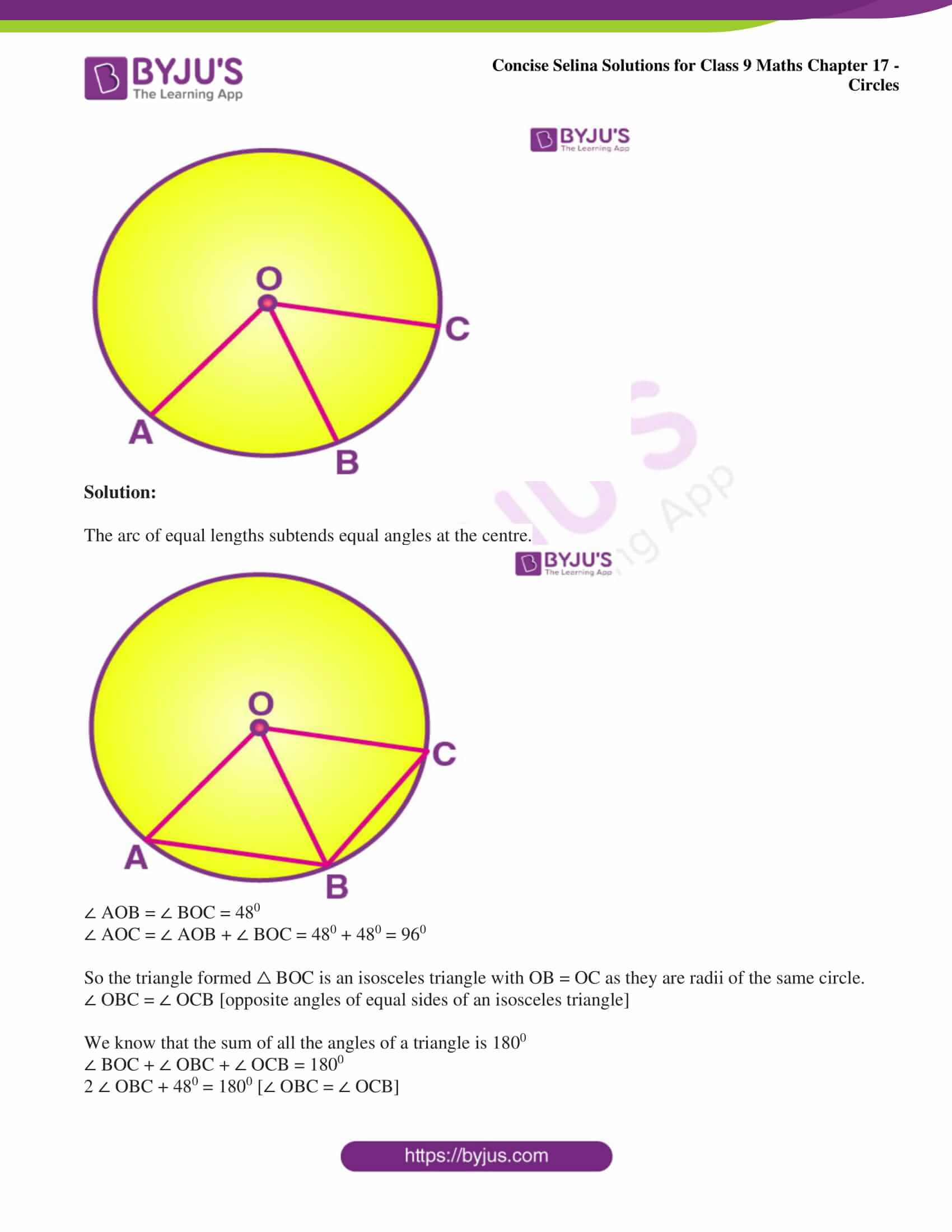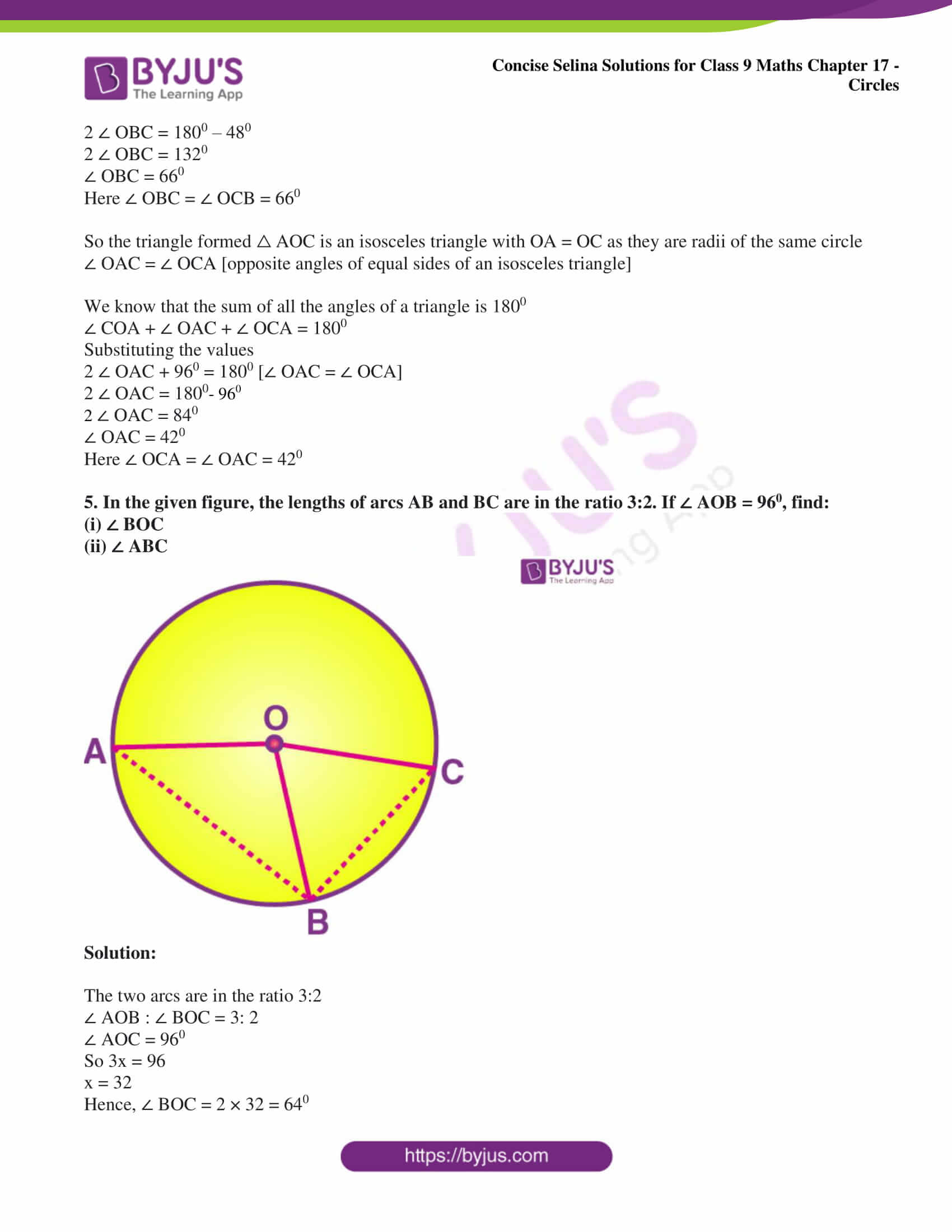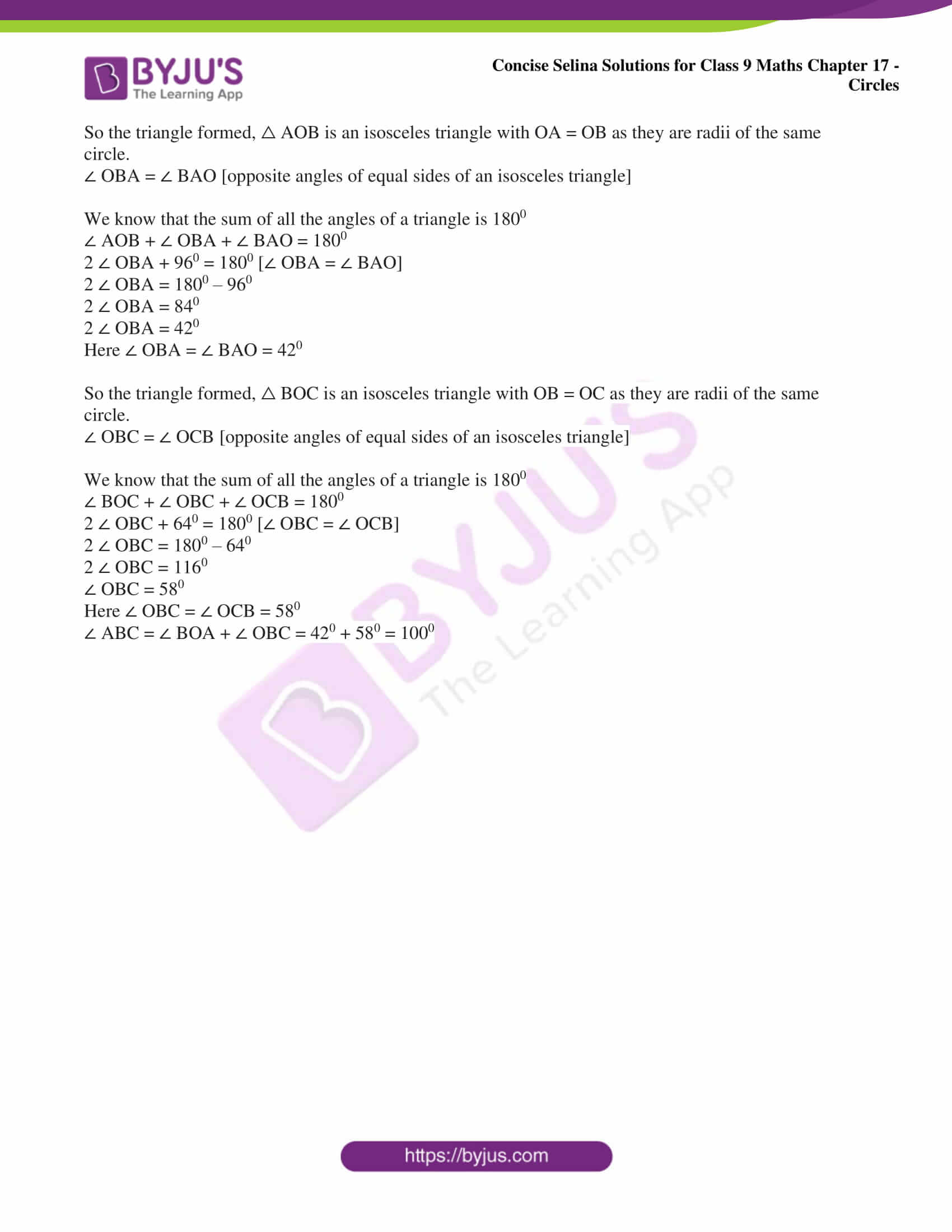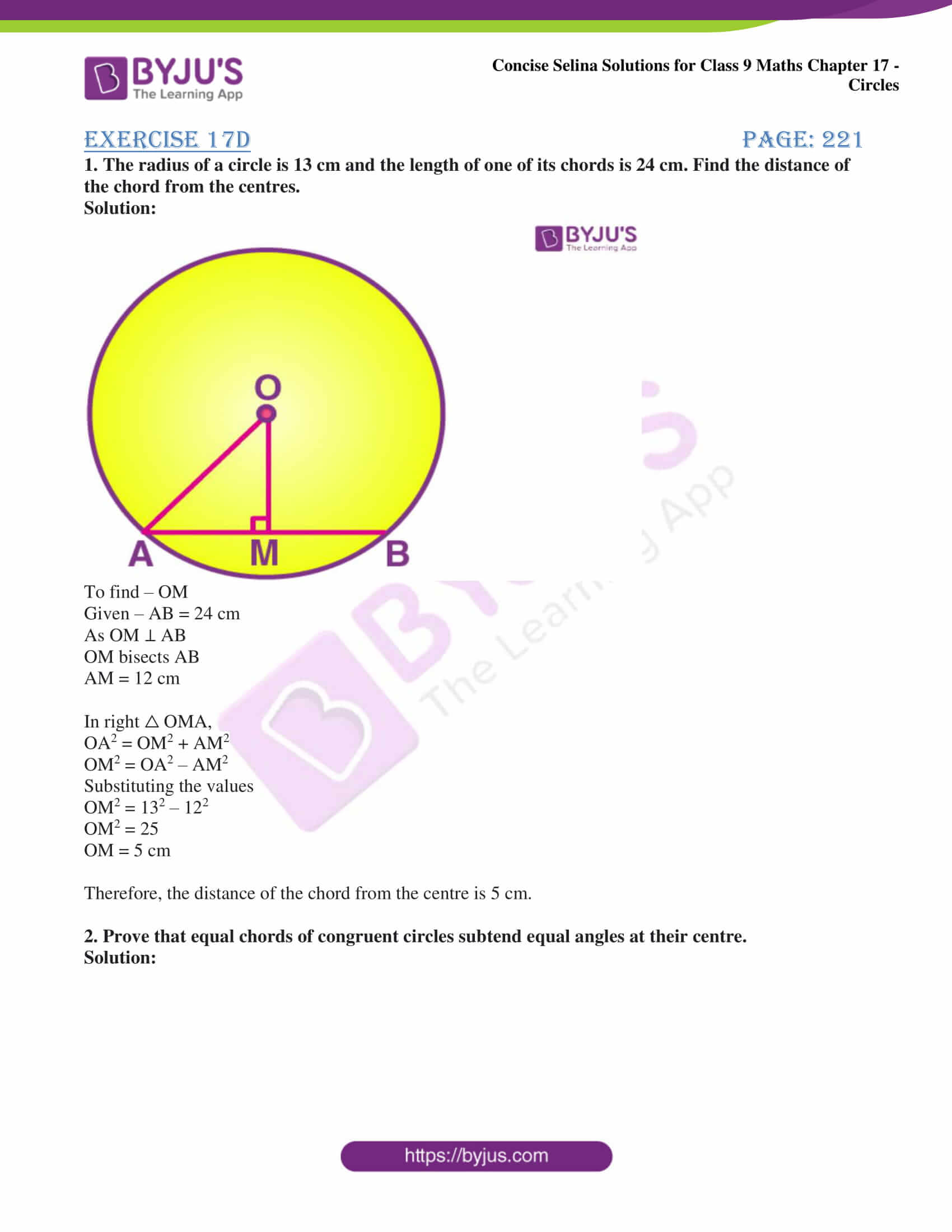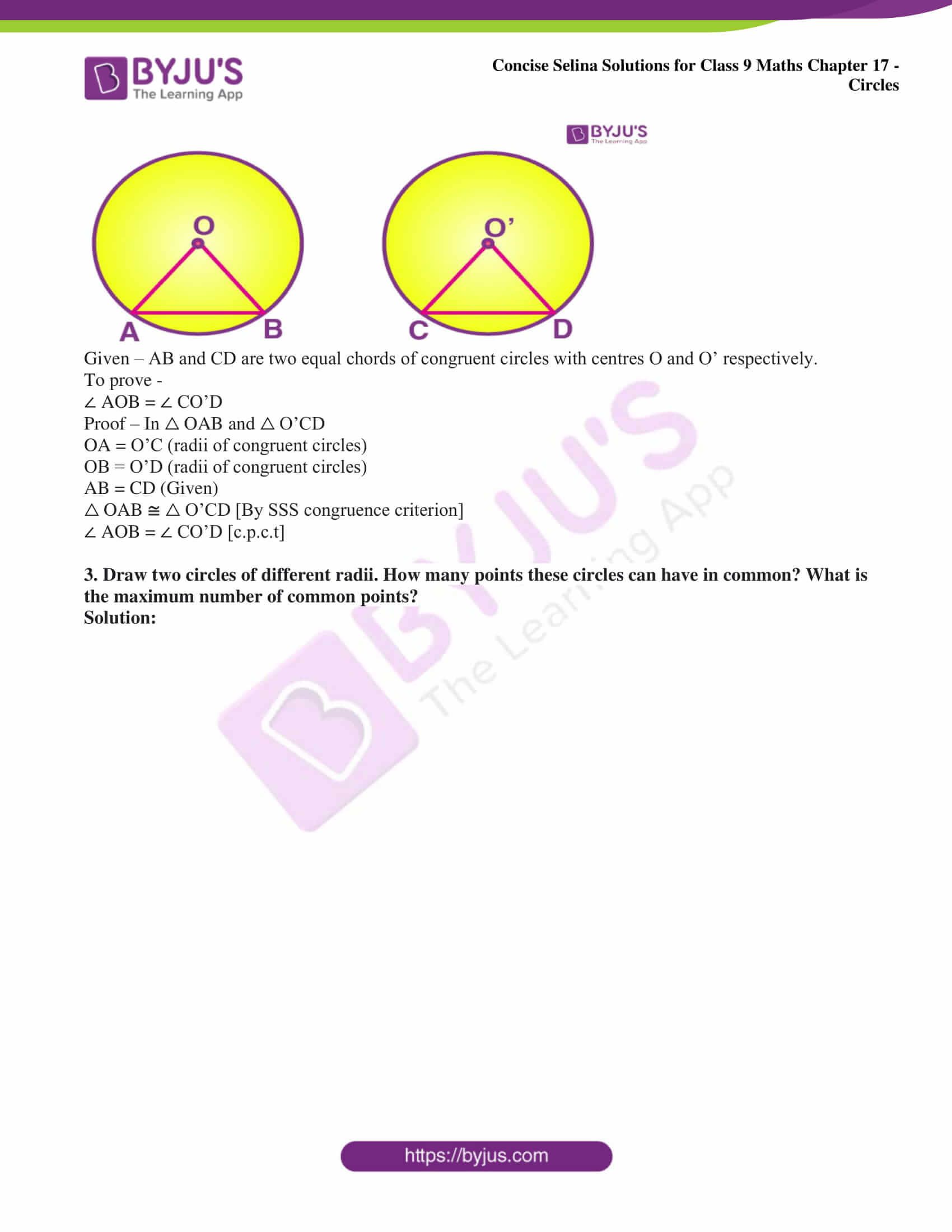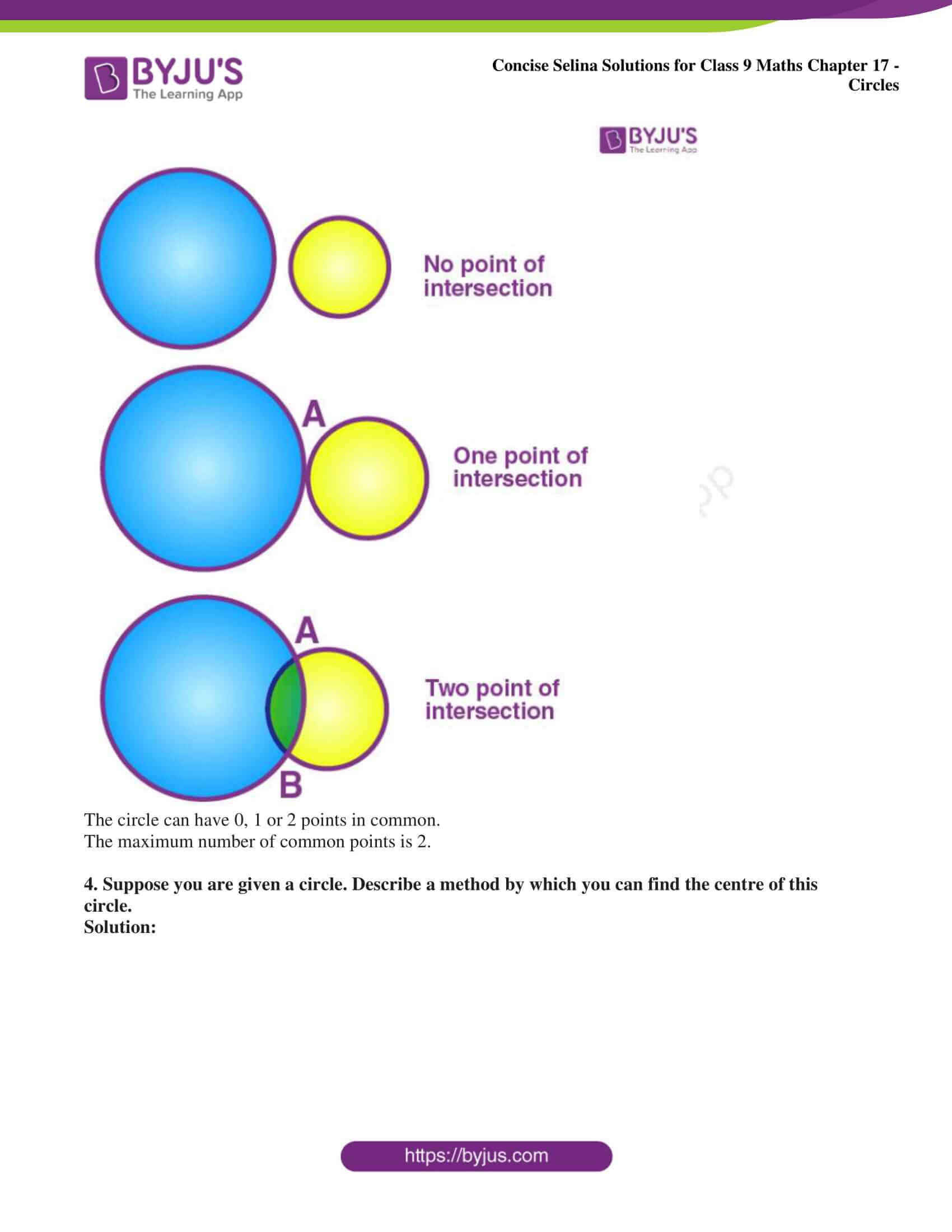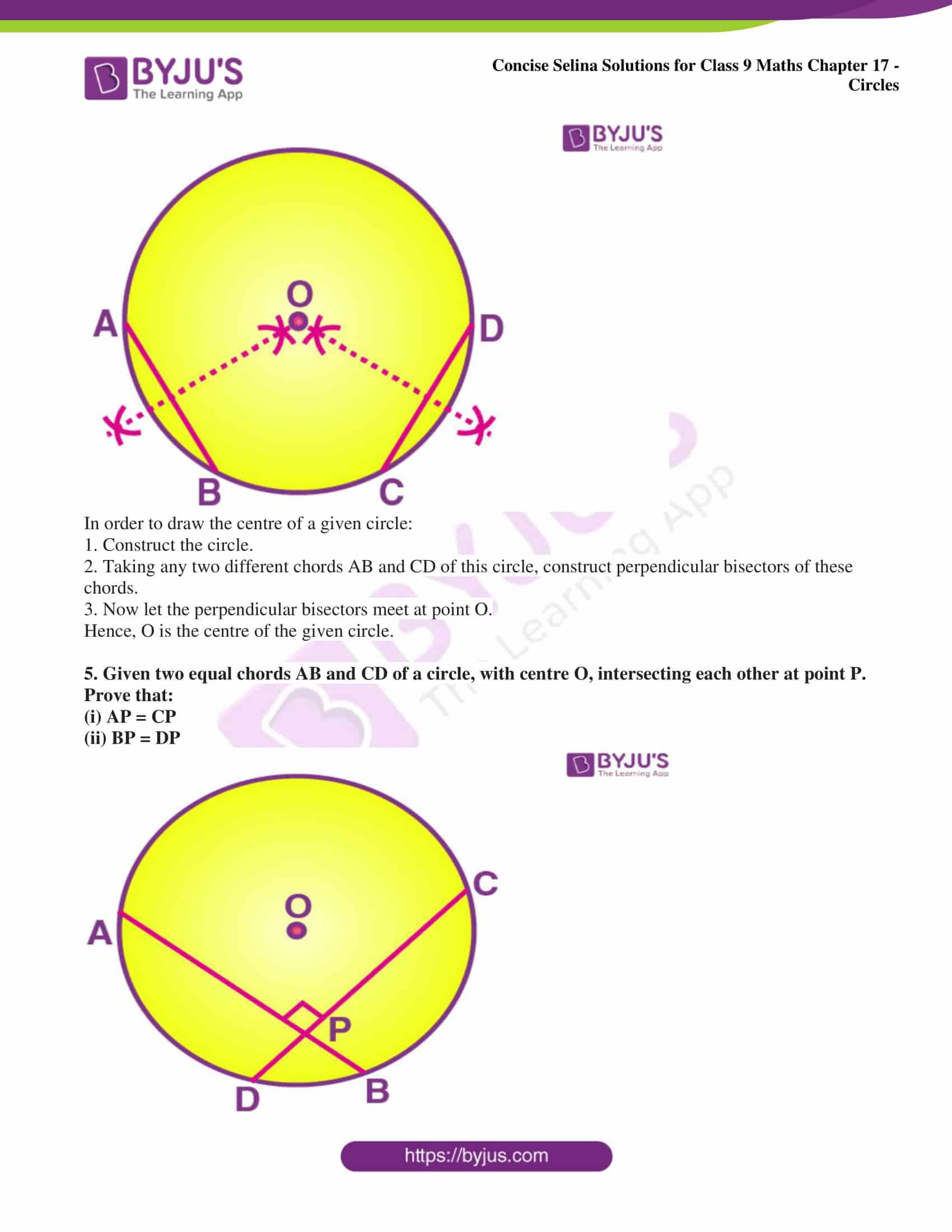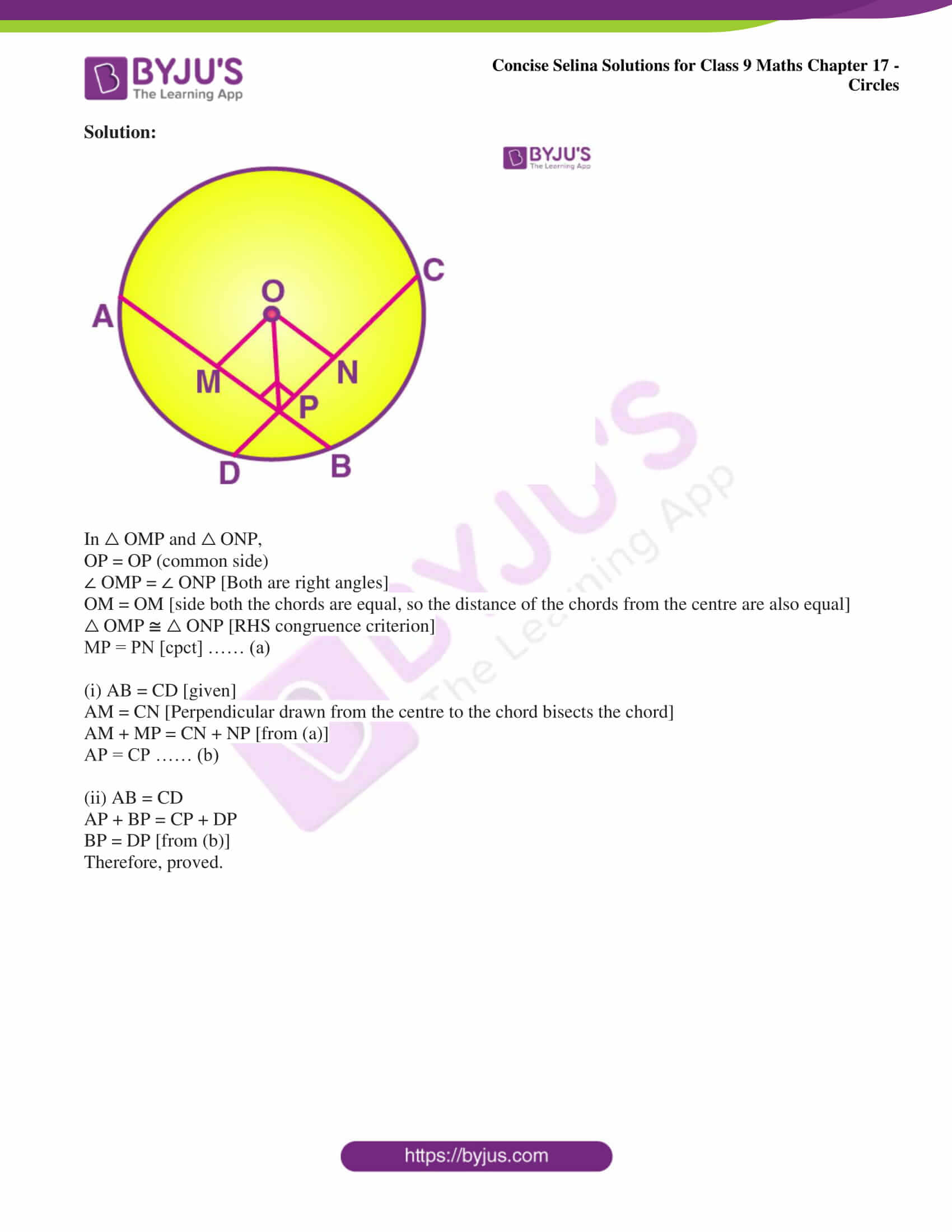Exercise 17A page: 210

1. A chord of length 6 cm is drawn in a circle of radius 5 cm. Calculate its distance from the centre of the circle.

Solution:

Consider AB as the chord and O as the centre of the circle.

Take OC as the perpendicular drawn from the centre O to AB.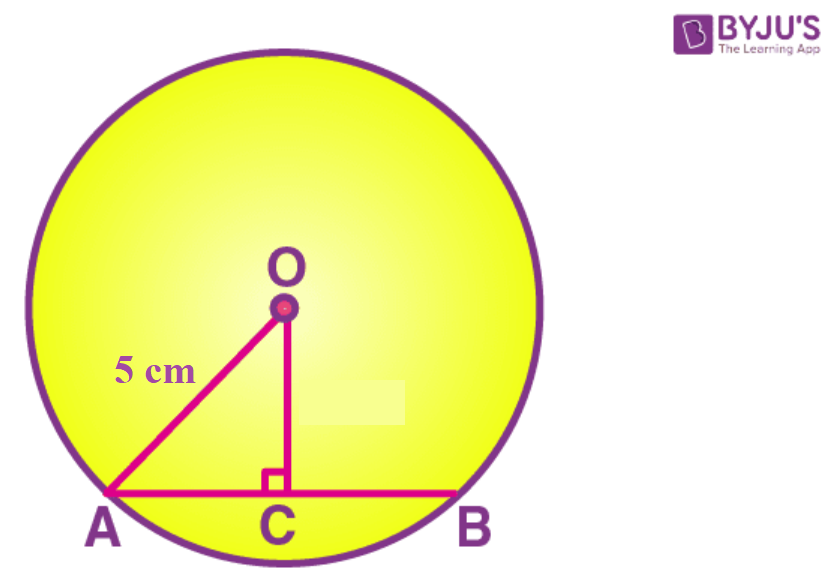Here, the perpendicular to a chord, from the centre of a circle, bisects the chord.

So, AC = CB = 3 cm

In △ OCA,

OA2 = OC2 + AC2 [Using Pythagoras Theorem]

Substituting the values

OC2 = 52 – 32

OC2 = 16

So we get

OC = 4 cm

2. A chord of length 8 cm is drawn at a distance of 3 cm from the centre of a circle. Calculate the radius of a circle.

Solution:

Consider AB as the chord and O as the centre of the circle.

Take OC as the perpendicular drawn from the centre O to AB.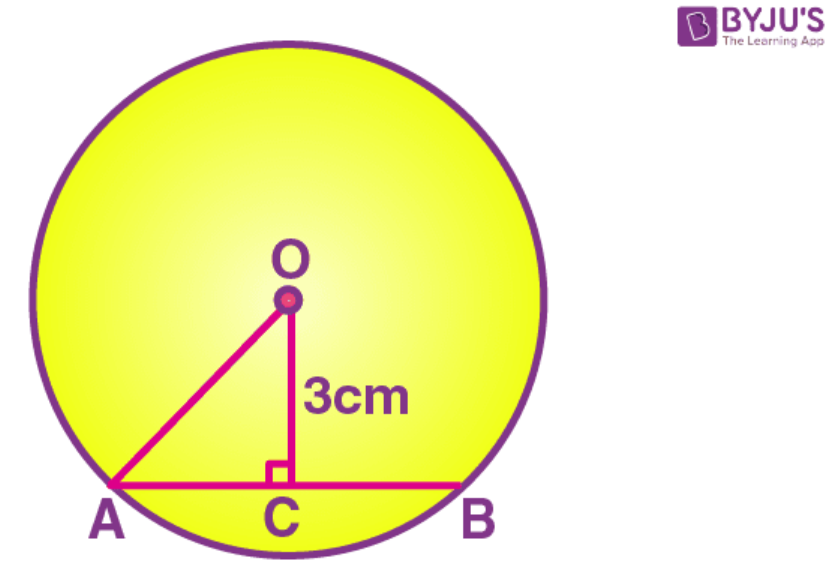Here, the perpendicular to a chord, from the centre of a circle, bisects the chord.

So, AB = 8 cm

We know that

AC = CB = AB/2

Substituting the value of AB

AC = CB = 8/2

AC = CB = 4 cm

In △ OCA,

OA2 = OC2 + AC2 [Using Pythagoras Theorem]

Substituting the values

OA2 = 42 + 32

OA = 25

So we get

OA = 5 cm

Therefore, radius of the circle is 5 cm.

3. The radius of a circle is 17.0 cm and the length of perpendicular is drawn from its center to a chord is 8.0 cm. Calculate the length of the chord.

Solution:

Consider AB as the chord and O as the centre of the circle.

Take OC as the perpendicular drawn from the centre O to AB.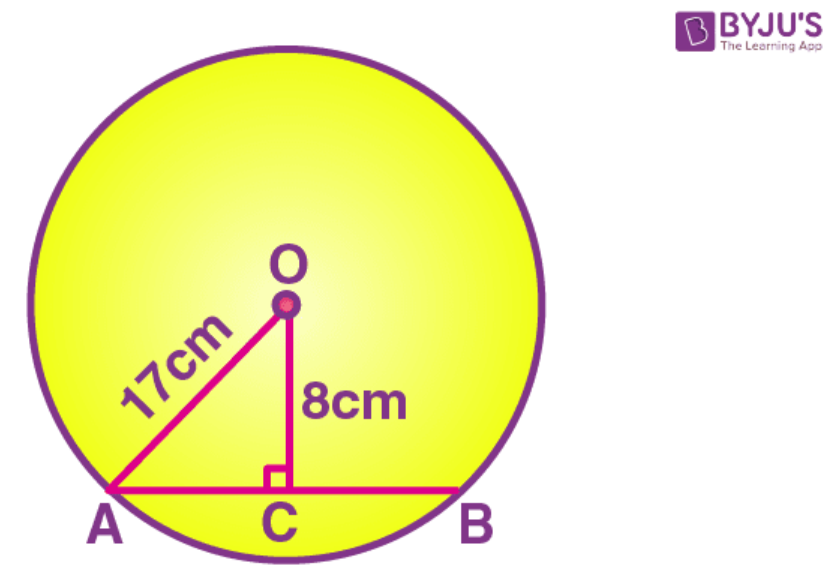Here, the perpendicular to a chord, from the centre of a circle, bisects the chord.

So, AC = CB

In △ OCA,

OA2 = OC2 + AC2 [Using Pythagoras Theorem]

Substituting the values

AC2 = 172 – 82

AC = 225

So we get

AC = 15 cm

AB = 2 AC = 2 × 15 = 30 cm

4. A chord of length 24 cm is at a distance of 5 cm from the centre of the circle. Find the length of the chord of the same circle which is at a distance of 12 cm from the centre.

Solution:

Consider AB as the chord of length 24 cm and O as the centre of the circle.

Take OC as the perpendicular drawn from the centre O to AB.

Here, the perpendicular to a chord, from the centre of a circle, bisects the chord.

So, AC = CB = 12 cm

In △ OCA,

OA2 = OC2 + AC2 [Using Pythagoras Theorem]

Substituting the values

OA2 = 52 + 122

OA = 169

So we get

OA = 13 cm

Therefore, radius of the circle is 13 cm.

Consider A’B’ as the new chord at a distance of 12 cm from the centre.

(OA’)2 = (OC’)2 + (A’C’)2

Substituting the values

(A’C’)2 = 132 – 122

(A’C’)2 = 25

A’C’ = 5 cm

Length of the new chord = 2 × 5 = 10 cm

5. In the following figure, AD is a straight line. OP ⊥ AD and O is the centre of both circles. If OA = 34 cm, OB = 20 cm and OP = 16 cm; find the length of AB.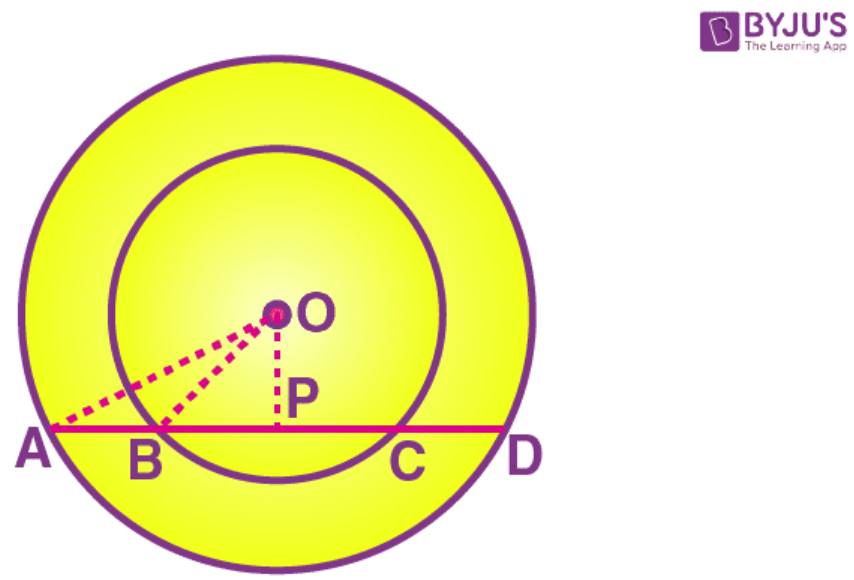Solution:In the inner circle, BC is the chord and OP ⊥ BC

Here, the perpendicular to a chord, from the centre of a circle, bisects the chord.

So, BP = PC

In △ OBP,

OB2 = OP2 + BP2 [Using Pythagoras Theorem]

Substituting the values

BP2 = 202 – 162

BP2 = 144

So we get

BP = 12 cm

In the outer circle, AD is the chord and OP ⊥ AD

Here, the perpendicular to a chord, from the centre of a circle, bisects the chord.

So, AP = PD

In △ OAP,

OA2 = OP2 + AP2 [Using Pythagoras Theorem]

Substituting the values

AP2 = 342 – 162

AP2 = 900

So we get

AP = 30 cm

Here, AB = AP – BP = 30 – 12 = 18 cm.

Exercise 17B PAGE: 217

1. The figure shows two concentric circles and AD is a chord of larger circle. Prove that: AB = CD.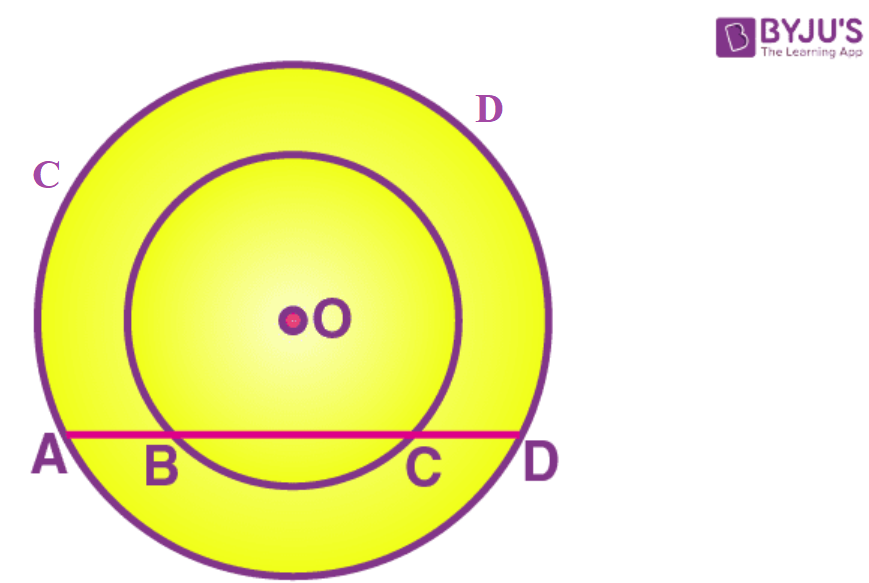Solution: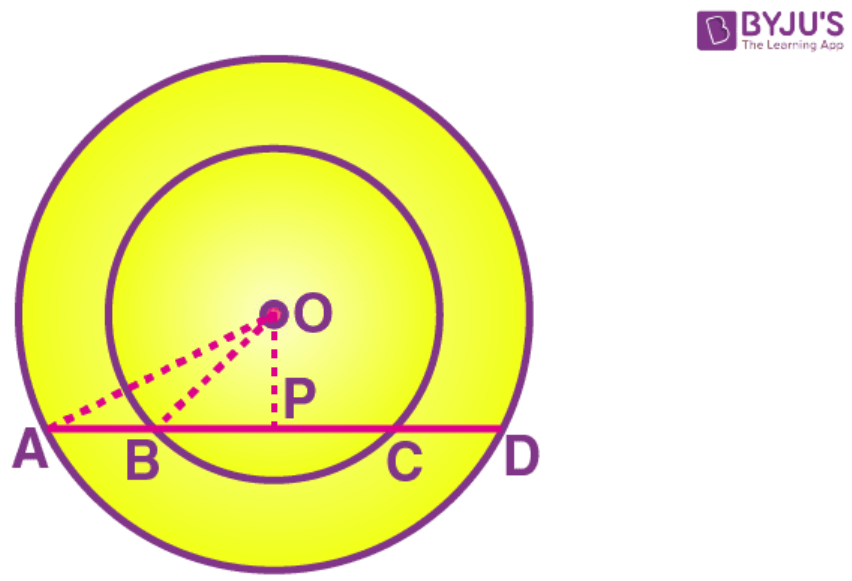[Perpendicular drawn from the centre of a circle to a chord bisects it.]

AP = PD ……… (i)

BC is a chord for the inner circle and OP ⊥ BC

So OP bisects BC

[Perpendicular drawn from the centre of a circle to a chord bisects it.]

BP = PC ………. (ii)

By subtracting equation (ii) from (i),

AP – BP = PD – PC

AB = CD

2. A straight line is drawn cutting two equal circles and passing through the midpoint M of the line joining their centres O and O’.

Prove that chords AB and CD, which are intercepted by the two circles are equal.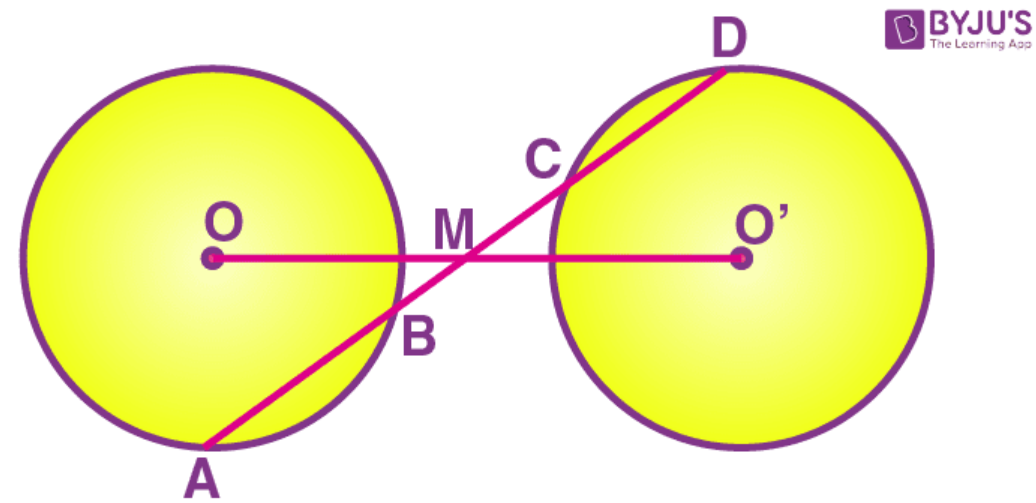Solution: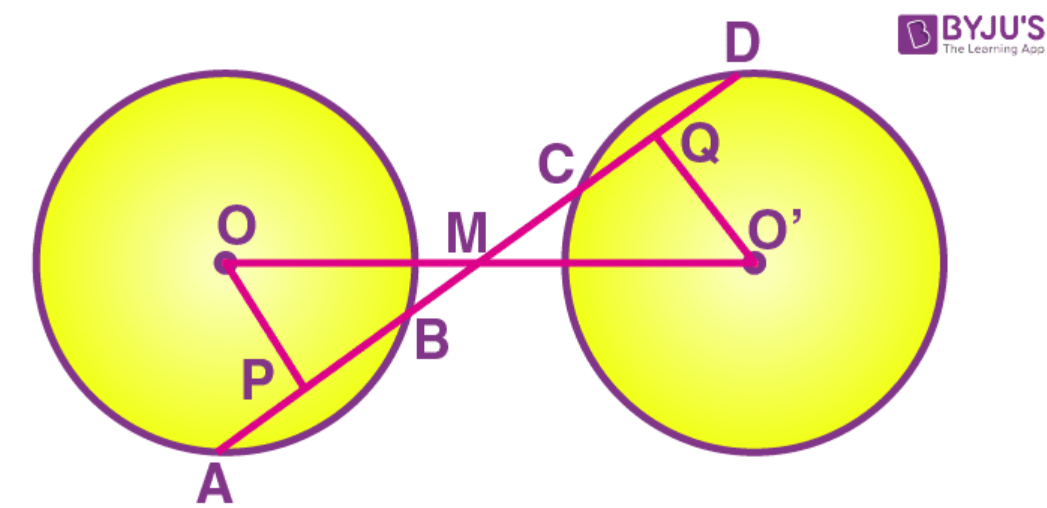Given –

A straight line AD intersects two circles of equal radii at A, B, C and D.

Line joining the centres OO’ intersect AD at M

M is the midpoint of OO’

To prove – AB = CD.

Construction – From the centre O, draw OP ⊥ AB and from O’ draw O’Q ⊥ CD.

Proof –

In △ OMP and △ O’MQ,

∠ OMP = ∠ O’MQ [vertically opposite angles]

∠ OPM = ∠ O’QM [each = 900]

OM = O’M [given]

By AAS criterion of congruence,

△ OMP △ O’MP

OP = O’Q [c.p.c.t]

Here, two chords of a circle or equal circles which are equidistant from the centre are equal.

AB = CD.

3. M and N are the mid-points of two equal chords AB and CD respectively of a circle with centre O. Prove that:

(i) ∠ BMN = ∠ DNM,

(ii) ∠ AMN = ∠ CNM.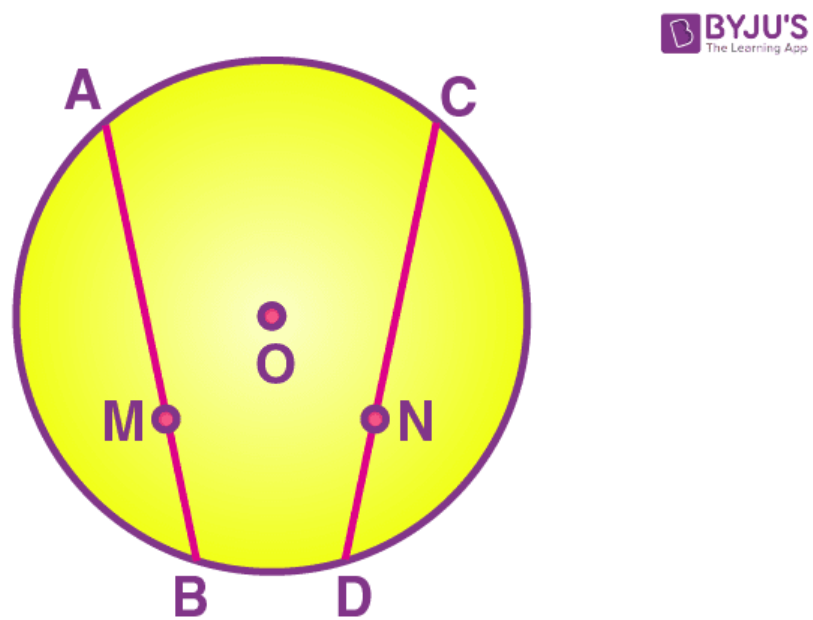Solution: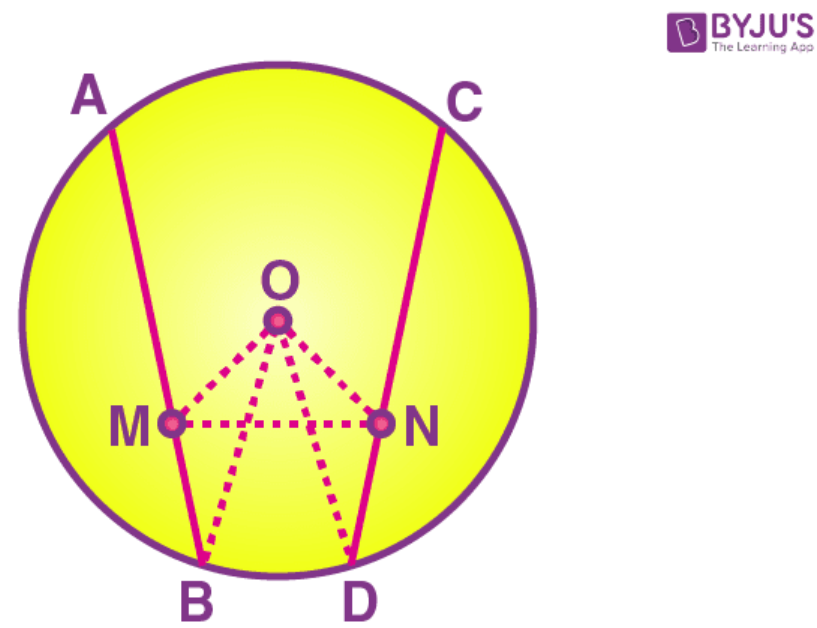Draw OM ⊥ AB and ON ⊥ CD

So OM bisects AB and ON bisects CD

[Perpendicular drawn from the centre of a circle to a chord bisects it.]

BM = ½ AB = ½ CD = DN ……..(1)

In △ OMB,

OM2 = OB2 + BM2 [Using Pythagoras Theorem]

We can write it as

OM2 = OD2 – DN2 [using equation (1)]

OM2 = ON2

OM = ON

So we get

∠ OMN = ∠ ONM ……. (2) [Angles opposite to the equal sides are equal]

(i) ∠ OMB = ∠ OND [both 900]

By subtracting (2) from above

∠ BMN = ∠ DNM

(ii) ∠ OMA = ∠ ONC [both 900]

∠ AMN = ∠ CNM

4. In the following figure: P and Q are the points of intersection of two circles with centres O and O’. If straight lines APB and CQD are parallel to OO’. Prove that

(i) OO’ = ½ AB

(ii) AB = CD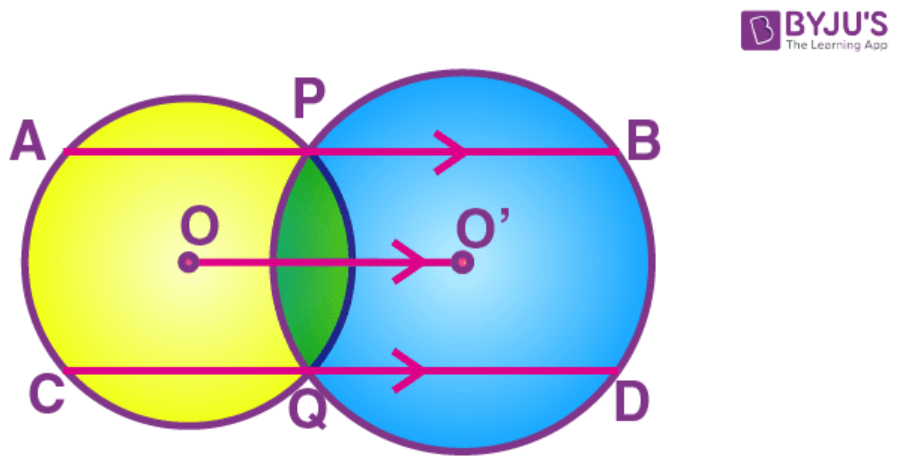Solution:

Draw OM and ON perpendicular on AB and OM’ and O’N’ perpendicular on CD.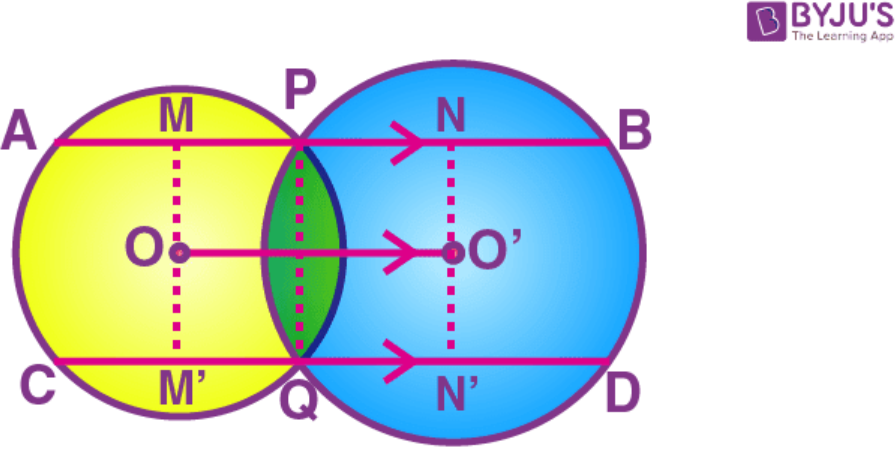So OM, O’N, OM’ and O’N’ bisect AP, PB, CQ and QD respectively

[Perpendicular drawn from the centre of a circle to a chord bisects it.]

MP = ½ AP, PN = ½ BP, M’Q = ½ CQ, QN’ = ½ QD

We know that

OO’ = MN = MP + PN = ½ (AP + BP) = ½ AB ……. (i)

OO’ = M’N’ = M’Q + QN’ = ½ (CQ + QD) = ½ CD …… (ii)

Equating (i) and (ii)

AB = CD

5. Two equal chords AB and CD of a circle with centre O, intersect each other at a point P inside the circle. Prove that:

(i) AP = CP

(ii) BP = DP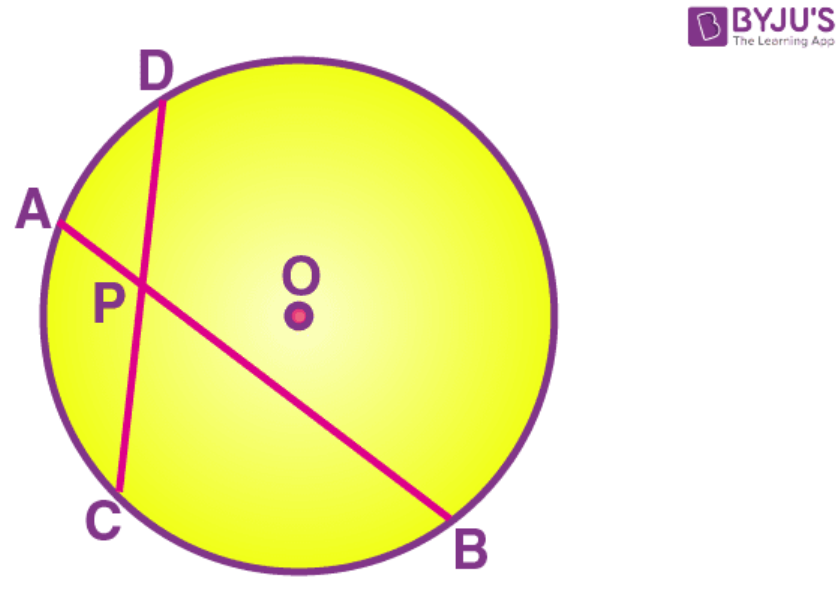Solution:

Draw OM and ON perpendicular on AB and CD.

Join OP, OB and OD.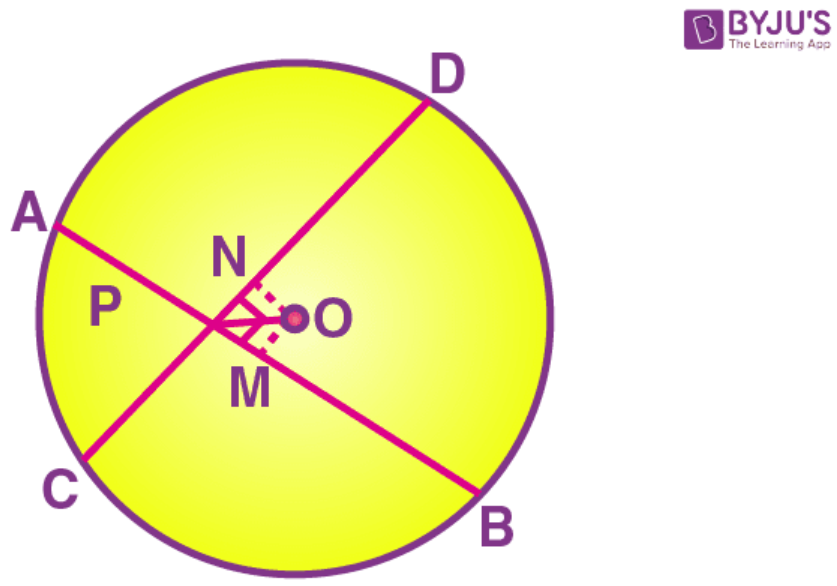So OM and ON bisect AB and CD respectively.

[Perpendicular drawn from the centre of a circle to a chord bisects it.]

MB = ½ AB = ½ CD = ND ……. (i)

In right triangle △ OMB,

OM2 = OB2 – MB2 ……. (ii)

In right triangle △ OND,

ON2 = OD2 – ND2 ……. (iii)

From equation (i), (ii) and (iii)

OM = ON

In △ OPM and △ OPN,

∠ OMP = ∠ ONP [both 900]

OP = OP [Common]

OM = ON [Proved]

Using RHS criterion of congruence,

△ OPM △ OPN

PM = PN [c.p.c.t]

MB + PM = ND + PN

BP = DP

We know that

AB = CD

AB – BP = CD – DP [BP = DP]

AP = CP

Exercise 17C PAGE: 220

1. In the given figure, an equilateral triangle ABC is inscribed in a circle with centre O. Find:

(i) ∠ BOC

(ii) ∠ OBC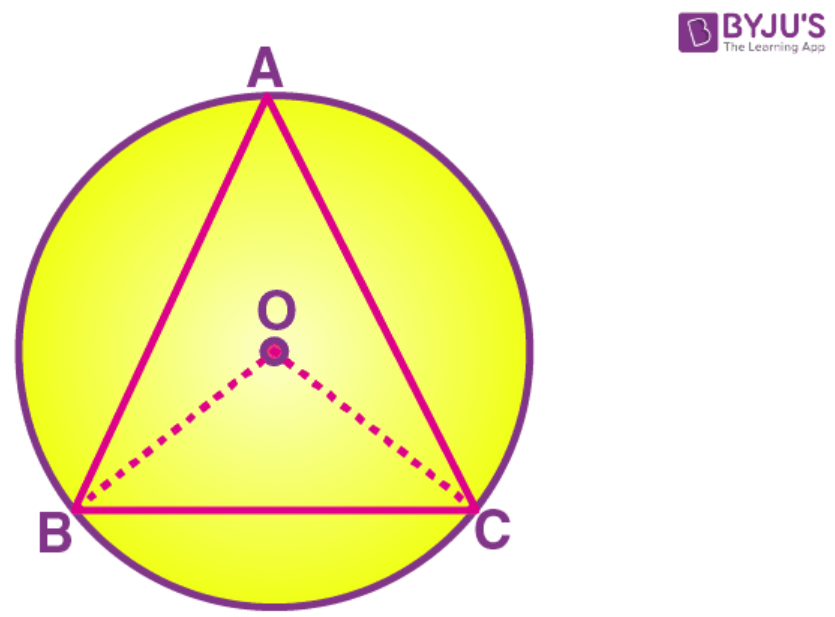Solution:

From the given figure, △ ABC is an equilateral triangle.

So all the three angles of the triangle will be 600.

∠ A = ∠ B = ∠ C = 600

As the triangle is equilateral, BO and CO will be the angle of bisectors of ∠ B and ∠ C respectively.

∠ OBC = ∠ ABC/2 = 300

From the given figure,

OB and OC are the radii of the given circle and are of equal length.

△ OBC is isosceles triangle with OB = OC.

In △ OBC,

∠ OBC = ∠ OCB as they are angles opposite to the two equal sides of an isosceles triangle.

∠ OBC = 300 and ∠ OCB = 300

As the sum of all the angles of a triangle is 1800

In △ OBC,

∠ OCB + ∠ OBC + ∠ BOC = 1800

Substituting the values

300 + 300 + ∠ BOC = 1800

600 + ∠ BOC = 1800

So we get

∠ BOC = 1800 – 600 = 1200

Therefore, ∠ BOC = 1200 and ∠ OBC = 300.

2. In the given figure, a square is inscribed in a circle with centre O. Find:

(i) ∠ BOC

(ii) ∠ OCB

(iii) ∠ COD

(iv) ∠ BOD

Is BD a diameter of the circle?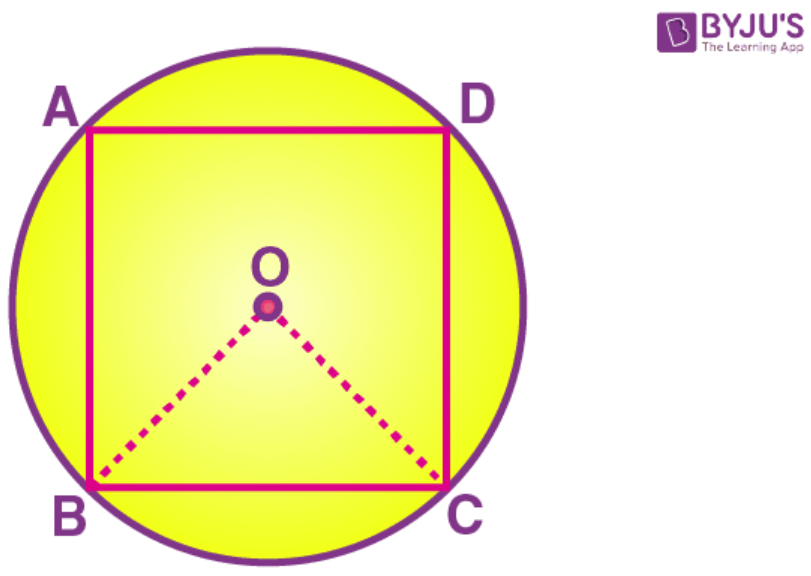Solution:

From the figure, extend a straight-line OB to BD and CO to CA.

We get the diagonals of the square which intersect each other at 900 by the property of square.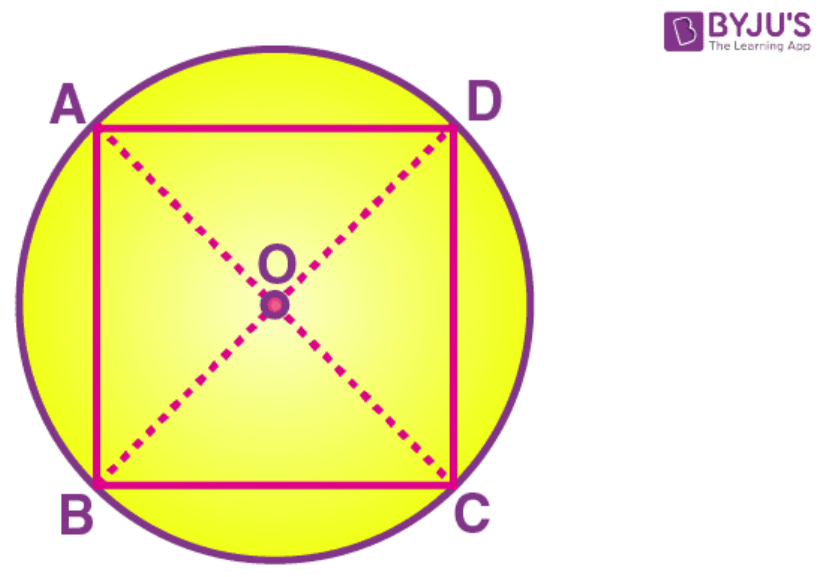From the above mentioned statement, we know that

∠ COD = 900

Here the sum of the angle ∠ BOC and ∠ OCD is 1800 as BD is a straight line.

∠ BOC + ∠ OCD = ∠ BOD = 1800

It can be written as

∠ BOC + 900 = 1800

∠ BOC = 1800 – 900

∠ BOC = 900

Therefore, triangle OCB is an isosceles triangle with sides OB and OC of equal length as they are the radii of the same circle.

In △ OCB,

∠ OBC = ∠ OCB [Opposite angles to the two equal sides of an isosceles triangle]

Here sum of all the angles of a triangle is 1800

∠ OBC + ∠ OCB + ∠ BOC = 1800

It can be written as

∠ OBC + ∠ OBC + 900 = 1800 [∠ OBC = ∠ OCB]

So we get

2 ∠ OBC = 1800 – 900

2 ∠ OBC = 900

∠ OBC = 450

Here, ∠ OBC = ∠ OCB = 450

Yes, BD is the diameter of the circle.

3. In the given figure, AB is a side of regular pentagon and BC is a side of regular hexagon.

(i) ∠ AOB

(ii) ∠ BOC

(iii) ∠ AOC

(iv) ∠ OBA

(v) ∠ OBC

(vi) ∠ ABC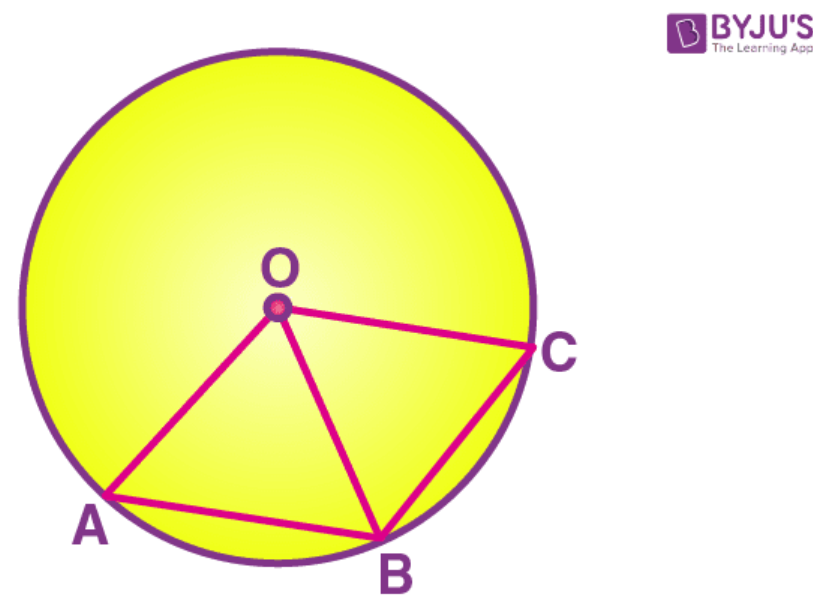Solution:

Given –

AB is the side of a pentagon where the angle subtended by each arm of the pentagon at the centre of the circle = 3600/5 = 720

Hence, ∠ AOB = 720

BC is the side of a hexagon where the angle subtended by BC at the centre = 3600/6 = 600

Hence, ∠ BOC = 600

∠ AOC = ∠ AOB + ∠ BOC

∠ AOC = 720 + 600 = 1320

The triangle formed i.e., △ AOB is an isosceles triangle with OA = OB as they are radii of the same circle.

∠ OBA = ∠ BAO [opposite angles of equal sides of an isosceles triangle]

We know that the sum of all the angles of a triangle is 1800

∠ AOB + ∠ OBA + ∠ BAO = 1800

2∠ OBA + 720 = 1800 [∠ OBA = ∠ BAO]

So we get

2 ∠ OBA = 1800 – 720

2 ∠ OBA = 1080

∠ OBA = 540

Here ∠ OBA = ∠ BAO = 540

So the triangle formed, △ BOC is an isosceles triangle with OB = OC as they are radii of the same circle.

∠ OBC = ∠ OCB [opposite angles of equal sides of an isosceles triangle]

We know that the sum of all the angles of a triangle is 1800

∠ BOC + ∠ OBC + ∠ OCB = 1800

Substituting the values

2 ∠ OBC + 600 = 1800 [∠ OBC = ∠ OCB]

2 ∠ OBC = 1800 – 600

2 ∠ OBC = 1200

∠ OBC = 600

Here ∠ OBC = ∠ OCB = 600

So ∠ ABC = ∠ OBA + ∠ OBC = 540 + 600= 1140

4. In the given figure, arc AB and arc BC are equal in length. If ∠ AOB = 480, find:

(i) ∠ BOC

(ii) ∠ OBC

(iii) ∠ AOC

(iv) ∠ OAC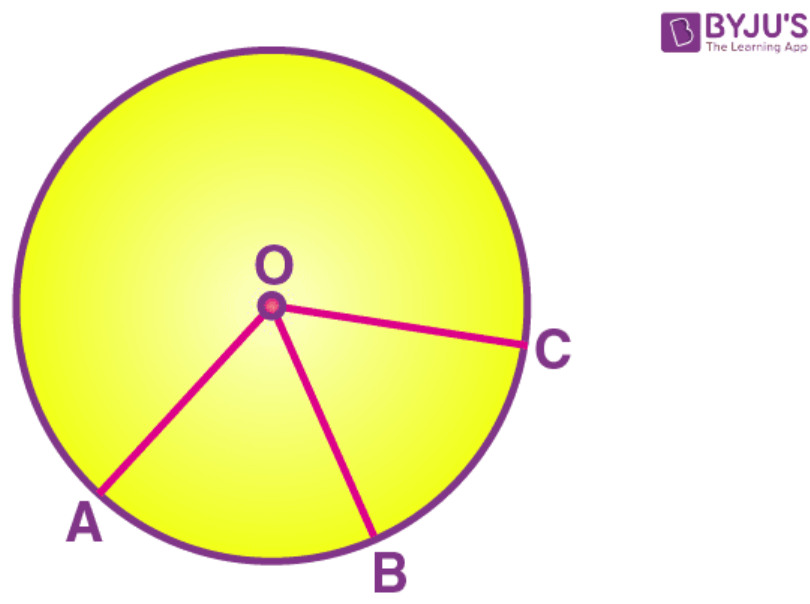Solution:

The arc of equal lengths subtends equal angles at the centre.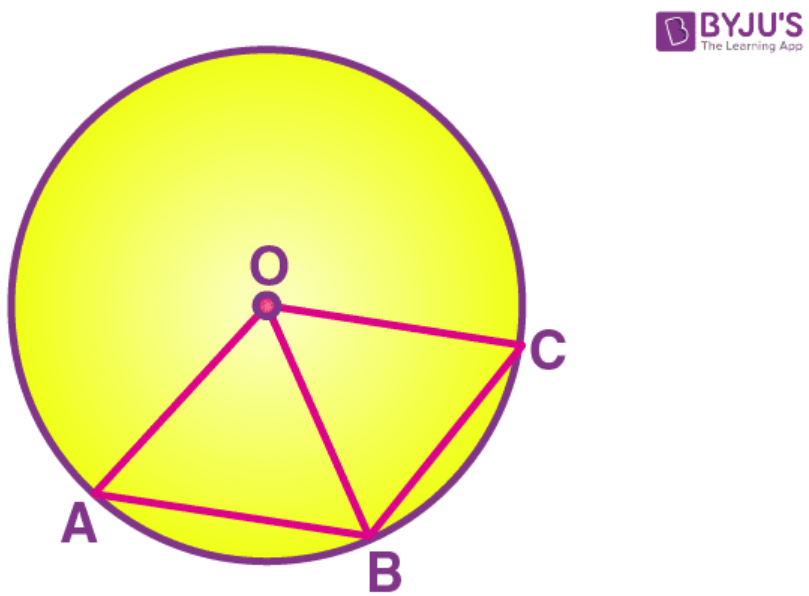∠ AOB = ∠ BOC = 480

∠ AOC = ∠ AOB + ∠ BOC = 480 + 480 = 960

So the triangle formed △ BOC is an isosceles triangle with OB = OC as they are radii of the same circle.

∠ OBC = ∠ OCB [opposite angles of equal sides of an isosceles triangle]

We know that the sum of all the angles of a triangle is 1800

∠ BOC + ∠ OBC + ∠ OCB = 1800

2 ∠ OBC + 480 = 1800 [∠ OBC = ∠ OCB]

2 ∠ OBC = 1800 – 480

2 ∠ OBC = 1320

∠ OBC = 660

Here ∠ OBC = ∠ OCB = 660

So the triangle formed △ AOC is an isosceles triangle with OA = OC as they are radii of the same circle

∠ OAC = ∠ OCA [opposite angles of equal sides of an isosceles triangle]

We know that the sum of all the angles of a triangle is 1800

∠ COA + ∠ OAC + ∠ OCA = 1800

Substituting the values

2 ∠ OAC + 960 = 1800 [∠ OAC = ∠ OCA]

2 ∠ OAC = 1800– 960

2 ∠ OAC = 840

∠ OAC = 420

Here ∠ OCA = ∠ OAC = 420

5. In the given figure, the lengths of arcs AB and BC are in the ratio 3:2. If ∠ AOB = 960, find:

(i) ∠ BOC

(ii) ∠ ABC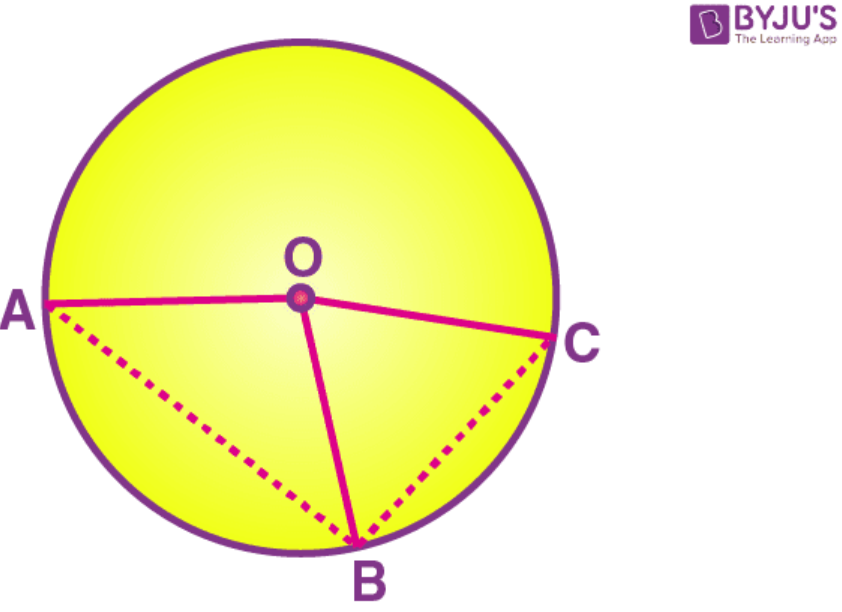Solution:

The two arcs are in the ratio 3:2

∠ AOB : ∠ BOC = 3: 2

∠ AOC = 960

So 3x = 96

x = 32

Hence, ∠ BOC = 2 × 32 = 640

So the triangle formed, △ AOB is an isosceles triangle with OA = OB as they are radii of the same circle.

∠ OBA = ∠ BAO [opposite angles of equal sides of an isosceles triangle]

We know that the sum of all the angles of a triangle is 1800

∠ AOB + ∠ OBA + ∠ BAO = 1800

2 ∠ OBA + 960 = 1800 [∠ OBA = ∠ BAO]

2 ∠ OBA = 1800 – 960

2 ∠ OBA = 840

2 ∠ OBA = 420

Here ∠ OBA = ∠ BAO = 420

So the triangle formed, △ BOC is an isosceles triangle with OB = OC as they are radii of the same circle.

∠ OBC = ∠ OCB [opposite angles of equal sides of an isosceles triangle]

We know that the sum of all the angles of a triangle is 1800

∠ BOC + ∠ OBC + ∠ OCB = 1800

2 ∠ OBC + 640 = 1800 [∠ OBC = ∠ OCB]

2 ∠ OBC = 1800 – 640

2 ∠ OBC = 1160

∠ OBC = 580

Here ∠ OBC = ∠ OCB = 580

∠ ABC = ∠ BOA + ∠ OBC = 420 + 580 = 1000

Exercise 17D PAGE: 221

1. The radius of a circle is 13 cm and the length of one of its chords is 24 cm. Find the distance of the chord from the centres.

Solution: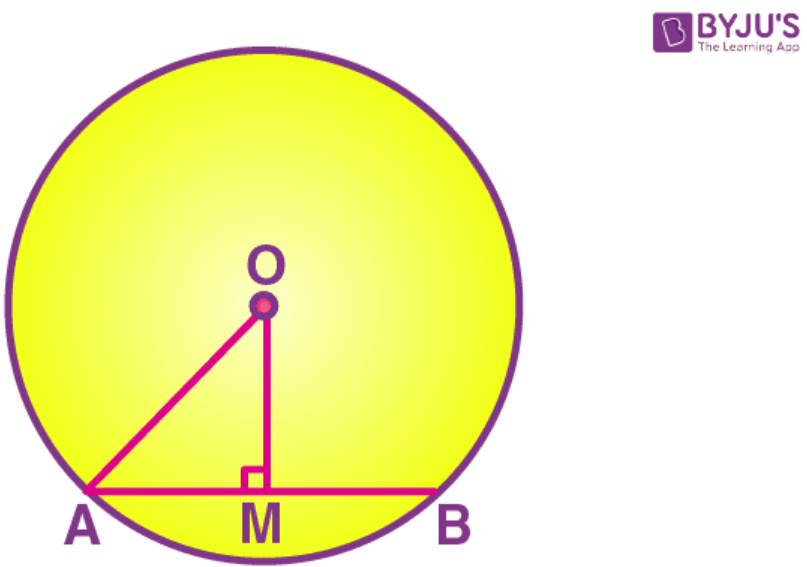To find – OM

Given – AB = 24 cm

As OM ⊥ AB

OM bisects AB

AM = 12 cm

In right △ OMA,

OA2 = OM2 + AM2

OM2 = OA2 – AM2

Substituting the values

OM2 = 132 – 122

OM2 = 25

OM = 5 cm

Therefore, the distance of the chord from the centre is 5 cm.

2. Prove that equal chords of congruent circles subtend equal angles at their centre.

Solution: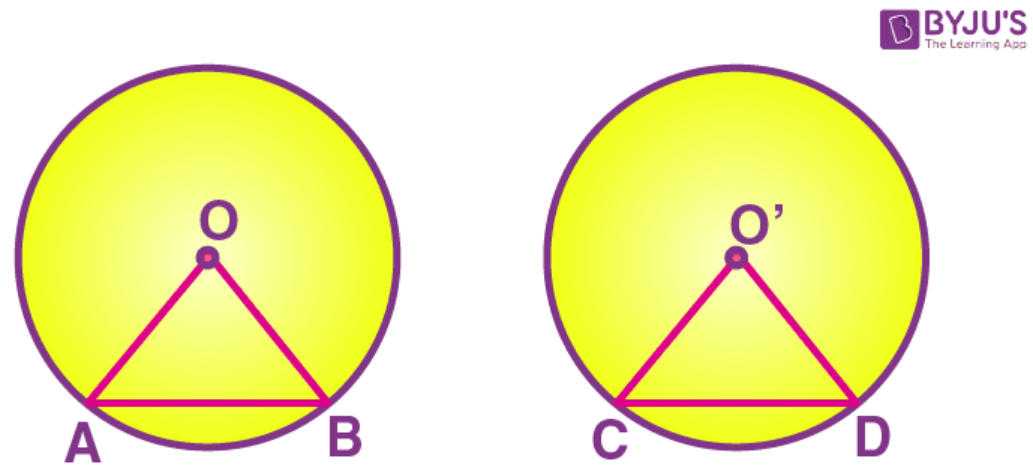Given – AB and CD are two equal chords of congruent circles with centres O and O’ respectively.

To prove –

∠ AOB = ∠ CO’D

Proof – In △ OAB and △ O’CD

OA = O’C (radii of congruent circles)

OB = O’D (radii of congruent circles)

AB = CD (Given)

△ OAB △ O’CD [By SSS congruence criterion]

∠ AOB = ∠ CO’D [c.p.c.t]

3. Draw two circles of different radii. How many points these circles can have in common? What is the maximum number of common points?

Solution: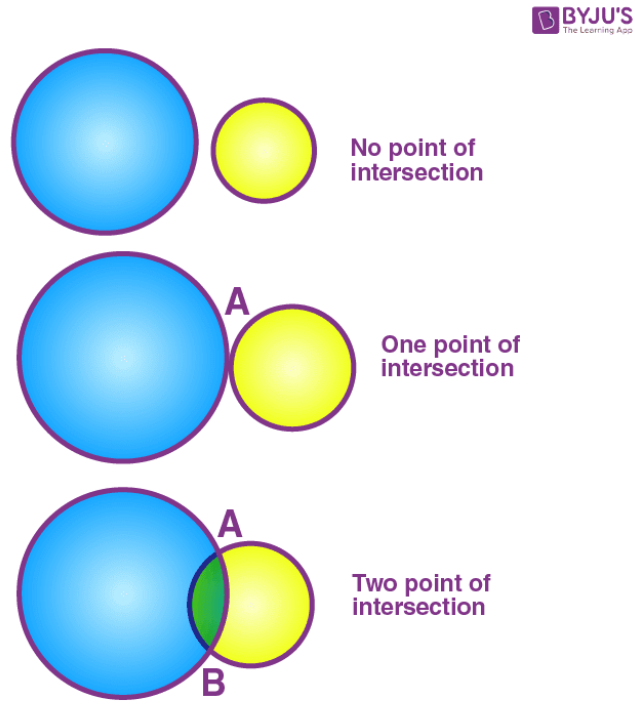The circle can have 0, 1 or 2 points in common.

The maximum number of common points is 2.

4. Suppose you are given a circle. Describe a method by which you can find the centre of this circle.

Solution: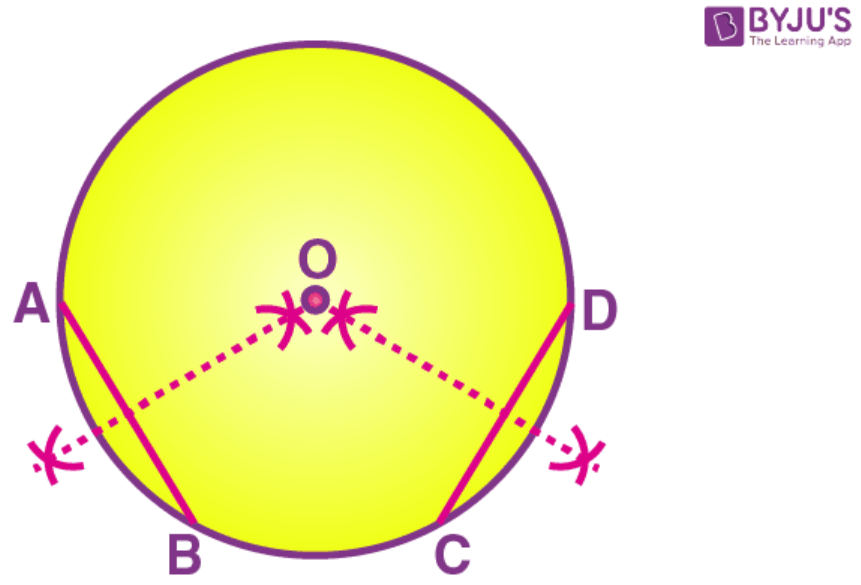In order to draw the centre of a given circle:

1. Construct the circle.

2. Taking any two different chords AB and CD of this circle, construct perpendicular bisectors of these chords.

3. Now let the perpendicular bisectors meet at point O.

Hence, O is the centre of the given circle.

5. Given two equal chords AB and CD of a circle, with centre O, intersecting each other at point P. Prove that:

(i) AP = CP

(ii) BP = DP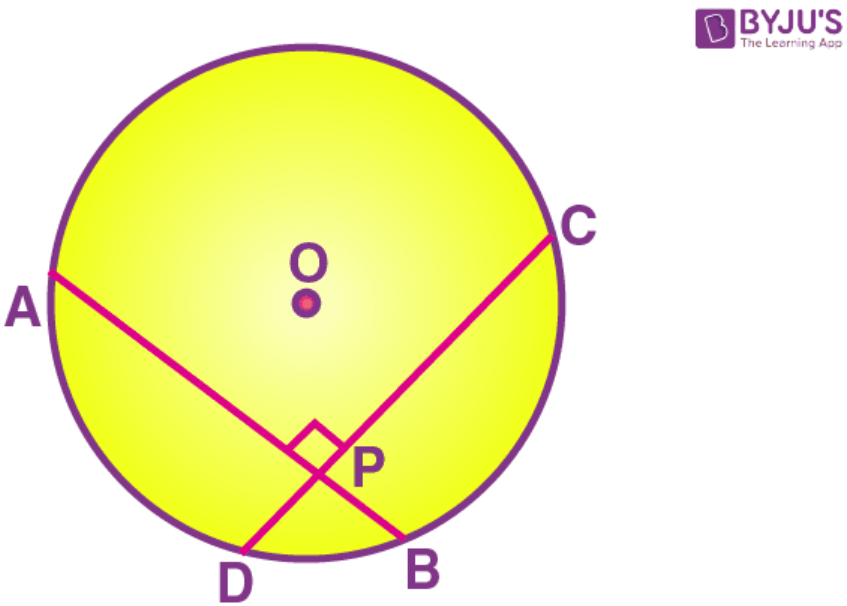Solution: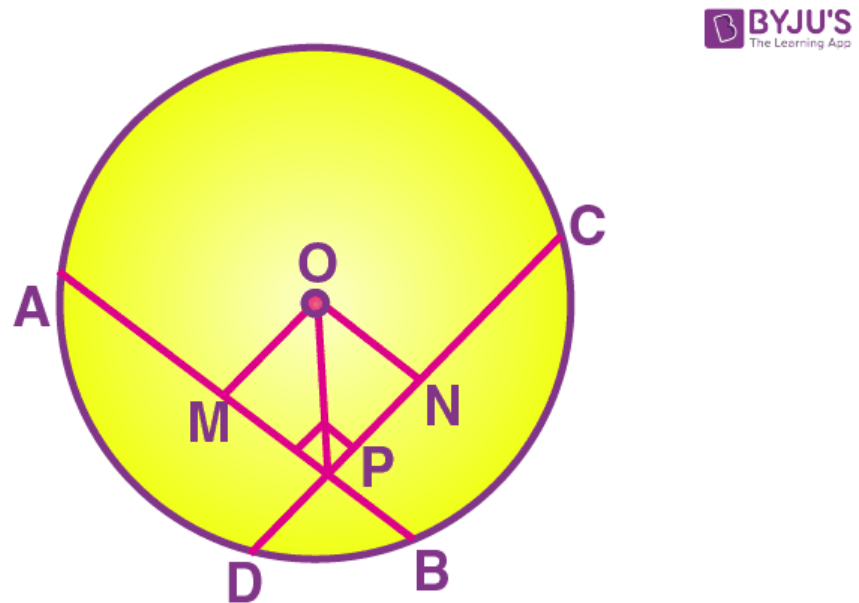In △ OMP and △ ONP,

OP = OP (common side)

∠ OMP = ∠ ONP [Both are right angles]

OM = OM [side both the chords are equal, so the distance of the chords from the centre are also equal]

△ OMP △ ONP [RHS congruence criterion]

MP = PN [cpct] …… (a)

(i) AB = CD [given]

AM = CN [Perpendicular drawn from the centre to the chord bisects the chord]

AM + MP = CN + NP [from (a)]

AP = CP …… (b)

(ii) AB = CD

AP + BP = CP + DP

BP = DP [from (b)]

Therefore, proved.

## Selina Solutions for Class 9 Maths Chapter 17- Circles

The Chapter 17, Circles, contains 4 exercises and the Solutions given here includes the answers for all the questions present in these exercises. Let us have a look at some of the topics that are being discussed in this chapter.

17.1 Introduction

17.2 Circle